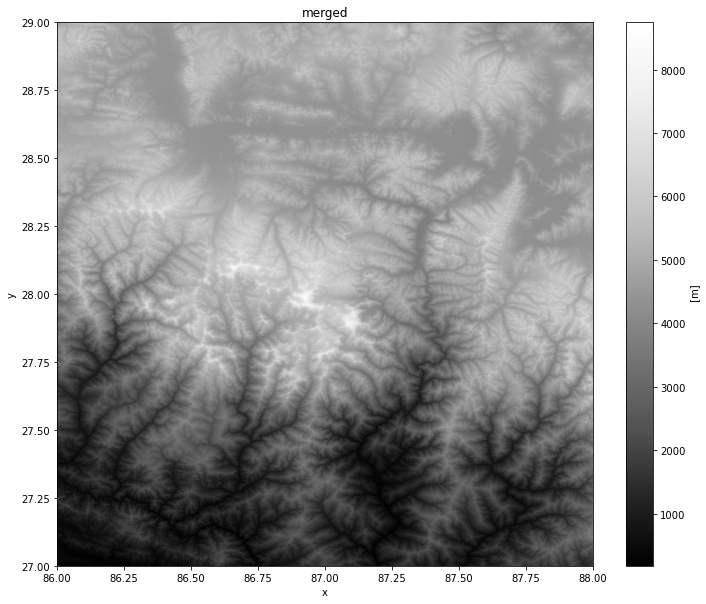安装和设置环境

1. 图形界面Graphical User Interface (GUI)：这就需要使用Anaconda Navigator 程序，有一个用户友好的界面，可以创建一个个独立的Python环境，以及安装依赖包。适合初学者。
2. 命令行界面Command Line Interface (CLI)：Anaconda提供了一个conda命令可以在终端（Windows的Command Prompt，macOS/Linux的Terminal）中运行。这个方法更快、效率更高，但需要对命令行有所了解。

图形界面 (Anaconda Navigator)

1. TUNA 提供了 Anaconda3 安装包 ，也可以访问 Anaconda 官网。一定注意要选择 Anaconda3，选择最新版本的，还要符合你的操作系统。（译者注：以 Windows 11 64bit 操作系统为例，就要选择 https://mirrors.tuna.tsinghua.edu.cn/anaconda/archive/Anaconda3-2021.11-Windows-x86_64.exe ；如果用的是 macOS，就可以选择 https://mirrors.tuna.tsinghua.edu.cn/anaconda/archive/Anaconda3-2021.11-MacOSX-x86_64.pkg ）。选最新版本是为了使用 Python 3.8 或更新的版本。下载好之后，双击安装，选择Just Me ，勾选use default settings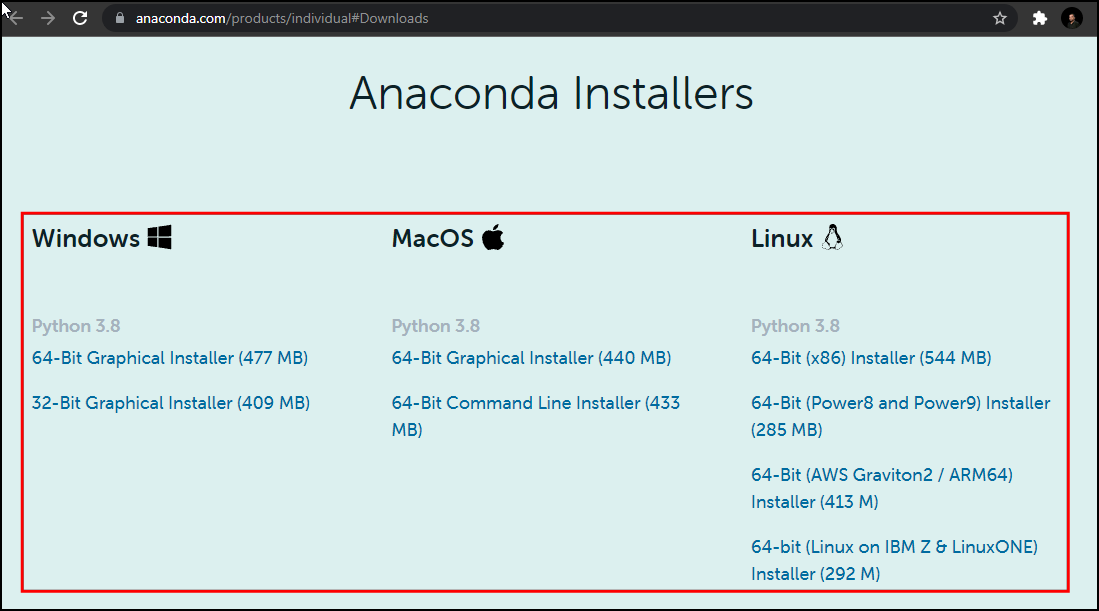1. 安装完成后，就可以启动Anaconda Navigator程序，在实践中，可以给你的每一个Python项目创造一个对应的单独的新环境environment。一个环境就是一个你用来安装各种依赖包的空间。很多包的依赖关系可能发生冲突，所以就不适合安装到一个单独的环境中去。分别创建环境就可以很好地规避这种问题。点击环境Environments 标签。

Linux用户需要打开终端输入anaconda-navigator来加载程序。1. 点击+ Create 按钮，然后输入环境名称为python_foundation。选中Python，设置版本为默认值就可以。然后点击创建Create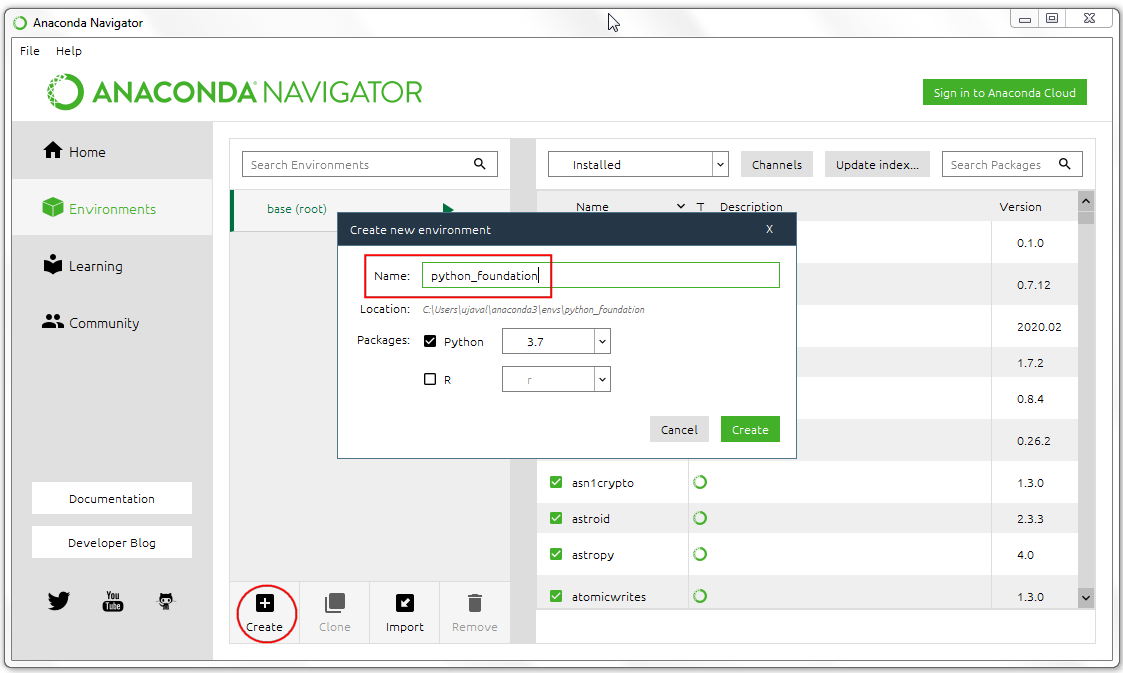1. 点击Update Channels按钮更新。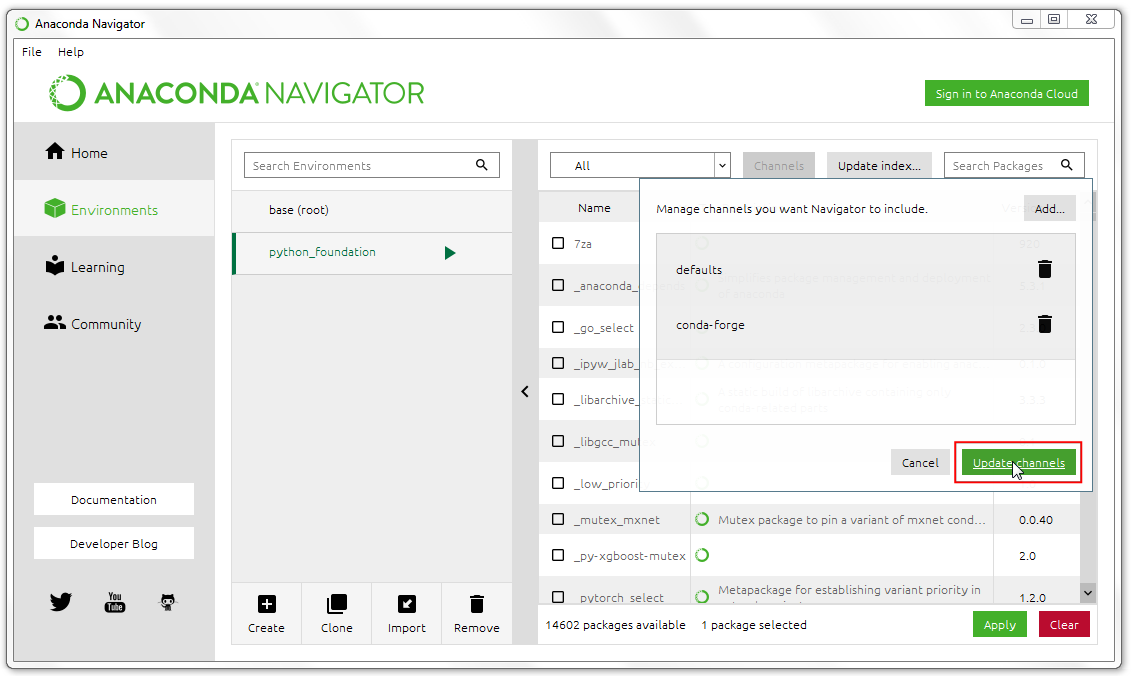1. 更新完成后，搜索一个名为 geopandas 的包。这个包适用于在Python中处理矢量地理空间数据进（vector geospatial data）。选中第一个搜索结果旁边的勾选框，然后点击应用Apply来安装所选包及其全部依赖。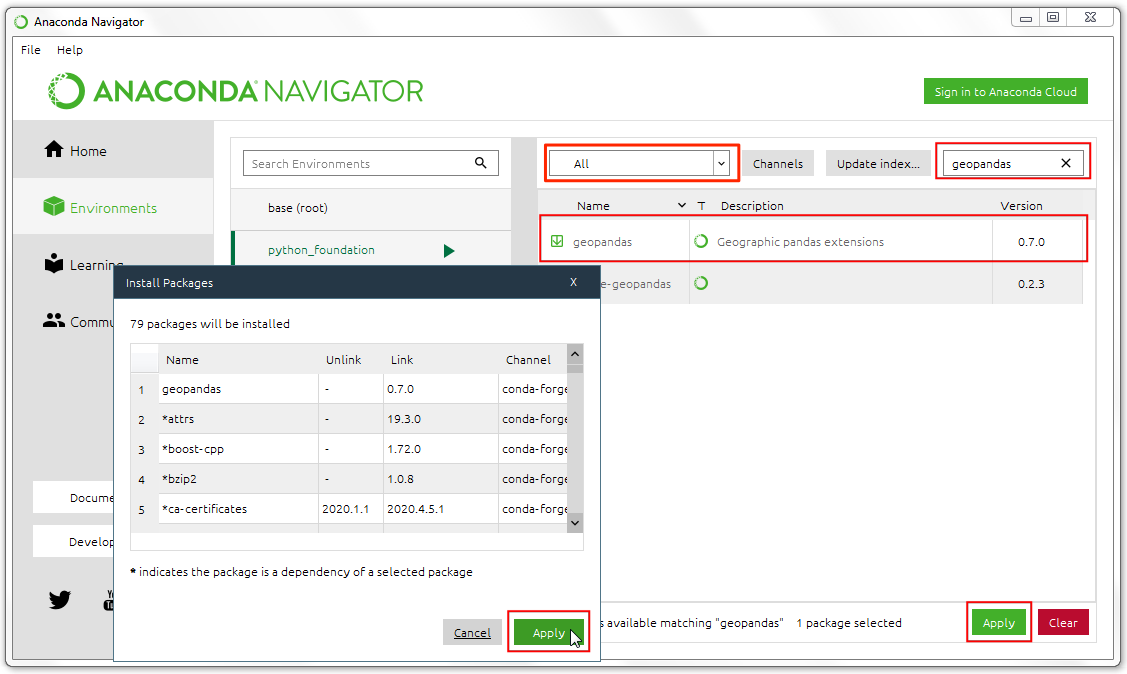1. 下面几个包也要用上面的步骤来安装。

2. geopy

3. rasterio
4. matplotlib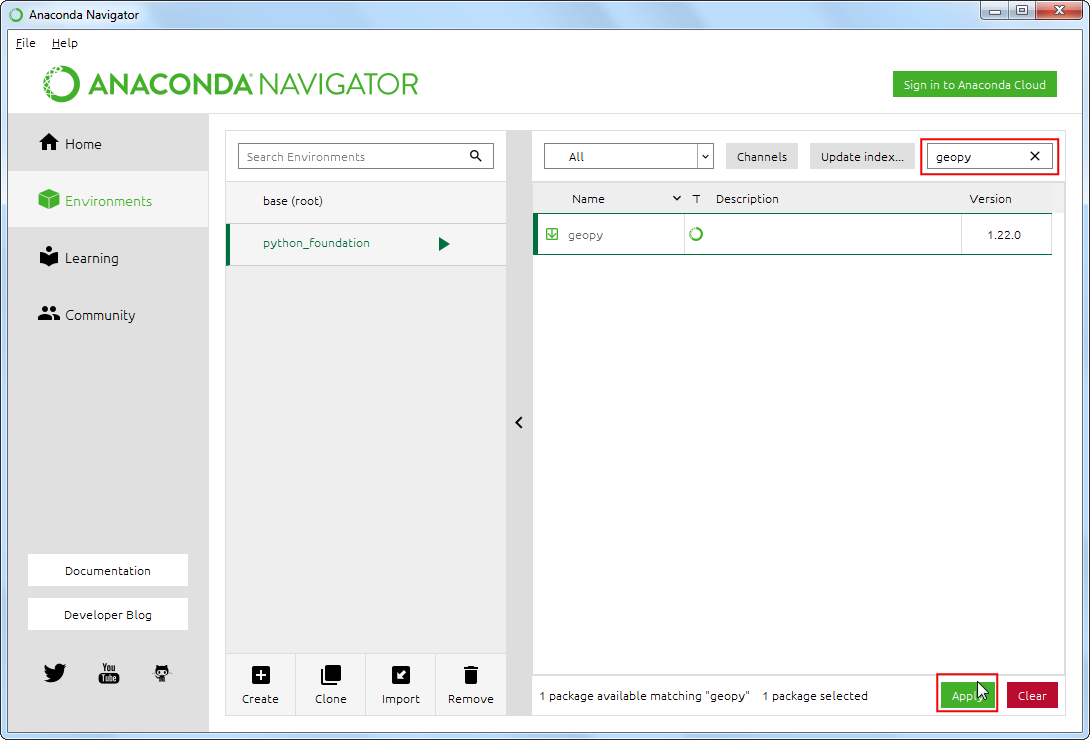1. 切换到Home标签页。确保你选中的事咱们刚创建的环境python_foundation 。接下来要安装几个程序来编写和运行Python脚本代码等等。在Home标签页上，选中安装JupyterLab这一程序。Windows系统用户可能需要安装CMD.exe prompt程序。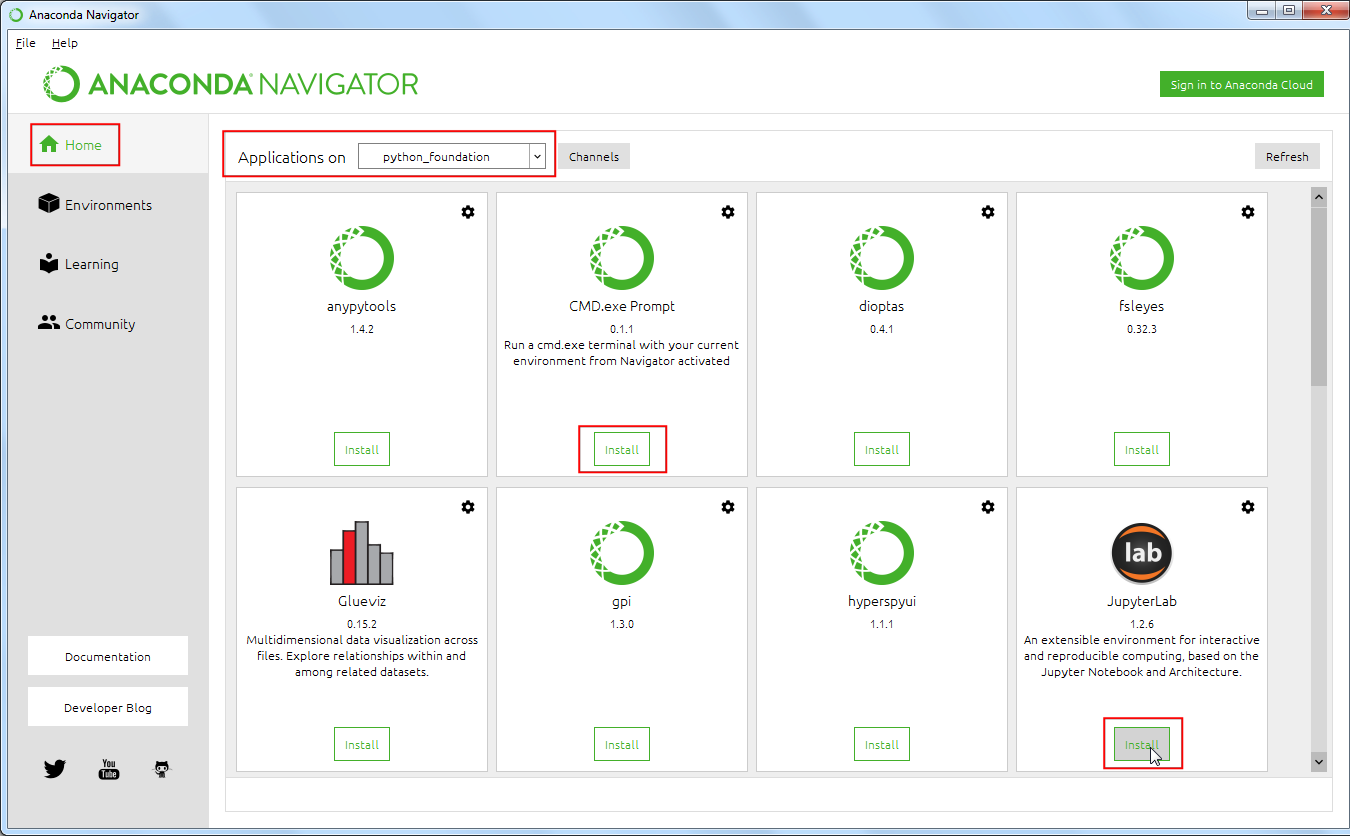1. 安装完成后，点击JupyterLab程序旁边的Launch 按钮来启动。JupyterLab是一个基于浏览器的应用，允许你来编写代码、文档以及运行代码。你可以在内部交互地运行一些小段代码。也支持图表、地图等等输出内容在界面上进行展示。这个程序的名字Jupyter来自于三种编程语言：Julia、Python、 R。本文的所有练习都使用Jupyter来进行。

Linux和macOS的命令行用户可以在终端中输入conda activate python_foundation来激活环境。然后终端中输入jupyter-lab命令就可以运行JupyterLab。1. 浏览器会弹出一个标签页，就是JupterLab的界面了。点击Notebook下面的Python 3按钮启动。1. 在第一个代码块（cell）中输入下面的代码，然后点击 Run 按钮来运行。如果什么都没有发狠过就证明你这几个依赖包的安装都成功了，没有任何问题。如果你遇到了导入错误 ImportError，就说明对应的包安装不成功，你得按照上面的教程重新安装一下。如果持续出现错误，就可能需要参考下一章的内容来使用命令行界面解决问题了。
import geopandas
import geopy
import rasterio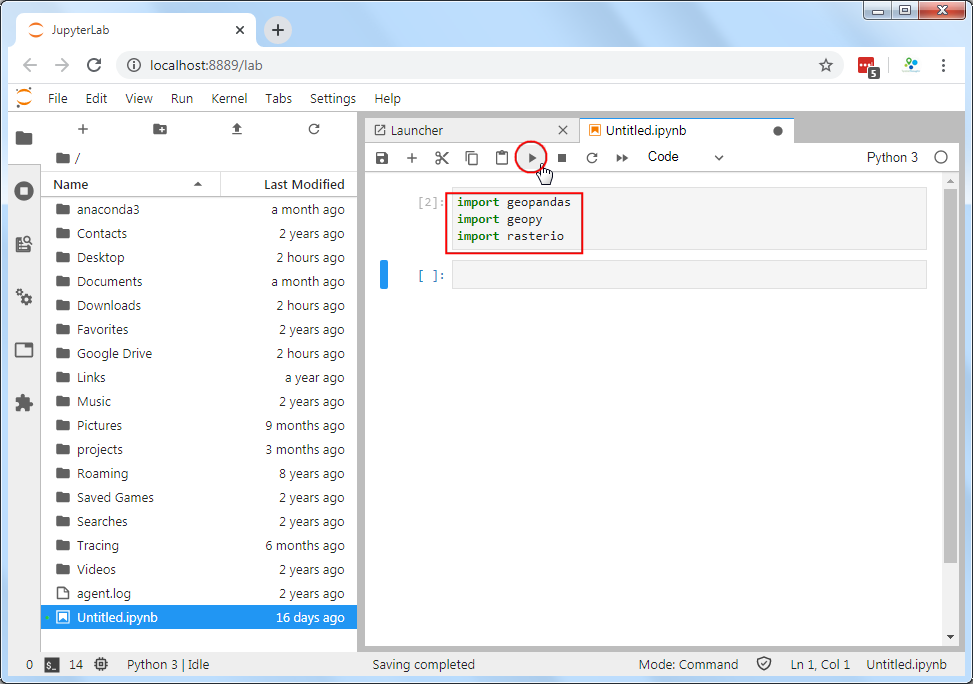命令行界面(Anaconda Prompt / Terminal)

1. 还是到 Anaconda3 官网下载安装包，版本还是要 Python3.8或者更高版本的，选对应你操作系统的版本。下载完成后还是选择Just Me以及使用默认设置use default settings1. 安装完成后，Windows用户从开始菜单找到并启动Anaconda Prompt 程序，macOS/Linux用户打开终端Terminal 。和上面一样，还是要为你的每个Python项目创建对应的独立的新环境。这个新环境就是用来存储这个Python项目对应的依赖包的。这样可以避免不同项目依赖包不同或者Python版本不同等因素导致的各种冲突和潜在问题。在命令行中输入下面的命令，回车执行。
conda create --name python_foundation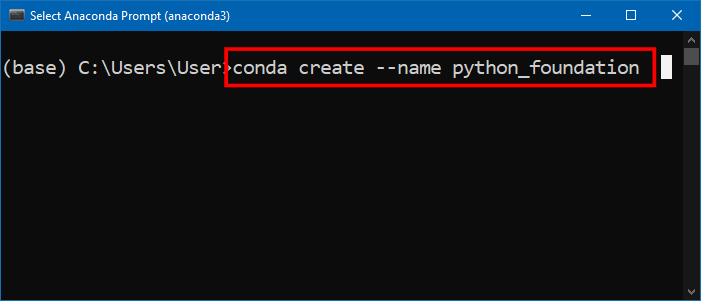1. 接下来会让你确认；输入y然后按回撤就继续完成环境创建了。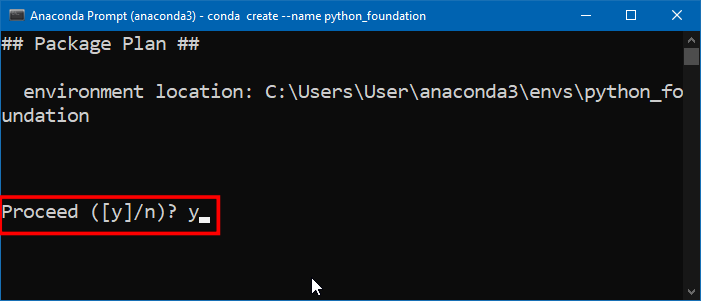1. 创建好环境之后还是要激活才生效，输入下面的命令然后按回车确认。激活之后，最前面的(base) 会变成(python_foundation)
conda activate python_foundation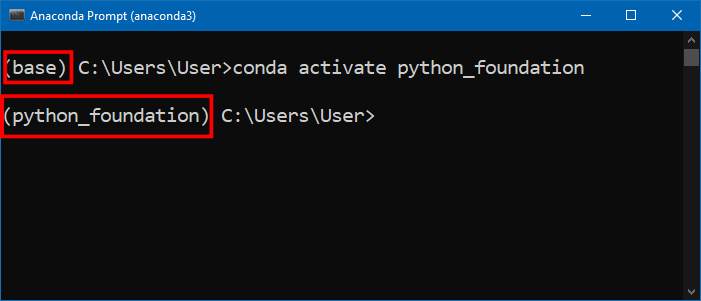1. 接下来就可以利用conda install命令来安装需要的包了。首先安装 geopandas，用来让Python能处理矢量化空间数据。需要使用conda-forge频道（channel）来安装这个包。后面的后缀-y 是用来确认，就跳过了它询问你是否继续哪一步来。输入命令后回车运行。
conda install --channel conda-forge geopandas -y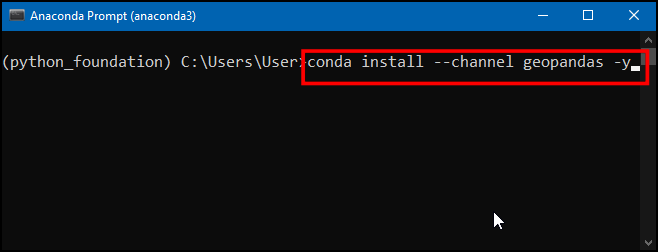1. 安装成功后，终端界面显示如下。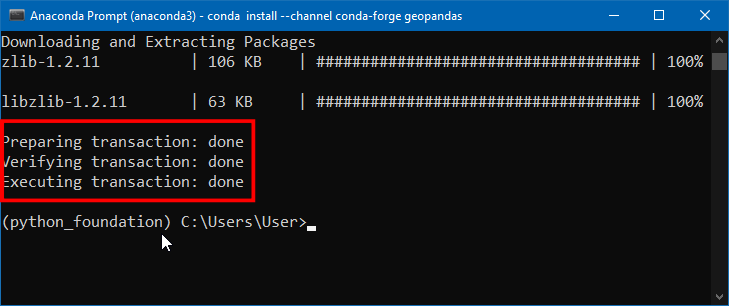1. geopandas 的安装还挺麻烦的，有时候可能不一定成功。所以安装完成后你可以用下面的命令来导入测试一下，看看有没有出错信息。python 命令后加一个 -c选项就是直接执行对应的语句，千万要注意这里要加英文双引号。输入命令后回车，没有任何错误信息输出就成功了。
python -c "import geopandas"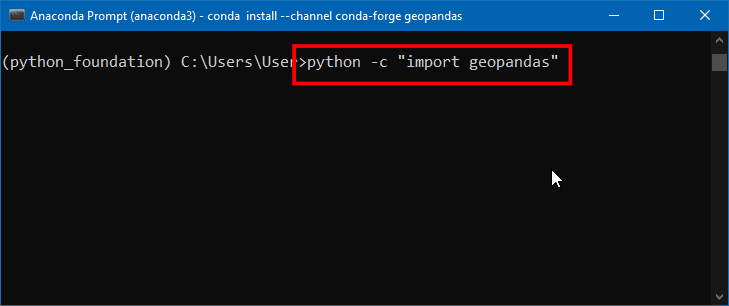1. 如果你的 geopandas 安装没问题，就什么输出都不会有。 什么都没发生，就说明你安装成功了。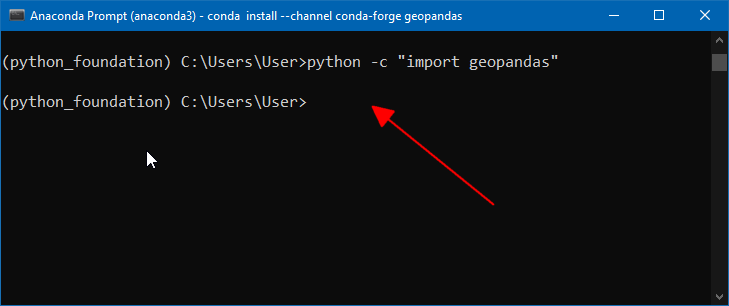1. 然后再安装其他的几个包， geopy, rasterio, matplotlibjupyterlab，安装命令如下，复制粘贴到终端，然后回车运行。
conda install --channel conda-forge geopy rasterio matplotlib jupyterlab -y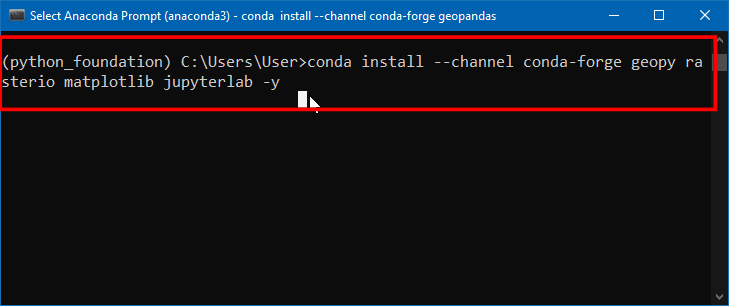1. 上述命令成功完成后，屏幕应该如下所示。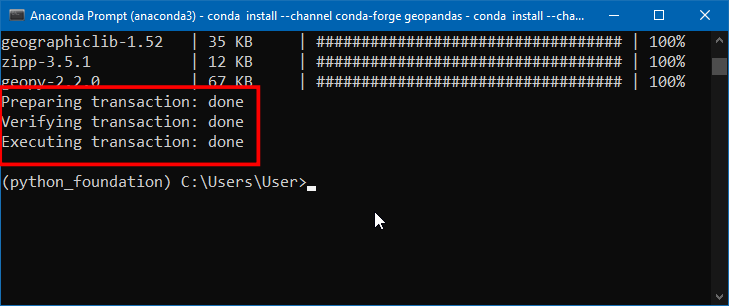1. 你的Python环境这就应该创建好了。在终端中输入下面的命令来启动JupyterLab就可以了。它会在你的系统上启动一个本地服务器，然后打开你的默认浏览器来访问JupyterLab的主界面。

jupyter-lab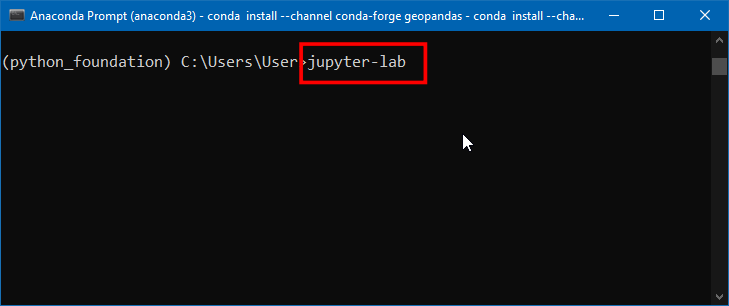12.浏览器页面会打开一个新标签页，就是JupyterLab的界面了。点击Notebook下面的Python 3 按钮。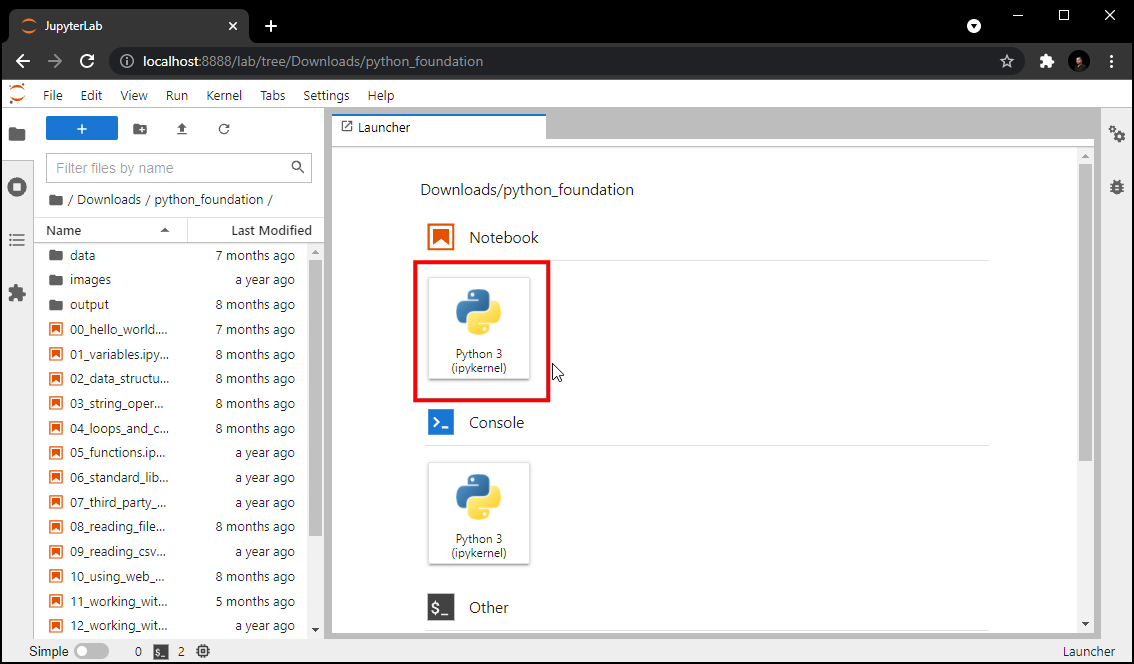1. 在第一个代码块（cell）里面输入下面的命令，然后点击小三角的那个 Run 运行按钮。 如果什么输出都没有，就说明安装成功了。如果你遇到导入错误ImportError，就说明安装未成功，重新安装吧。
import geopandas
import geopy
import rasterio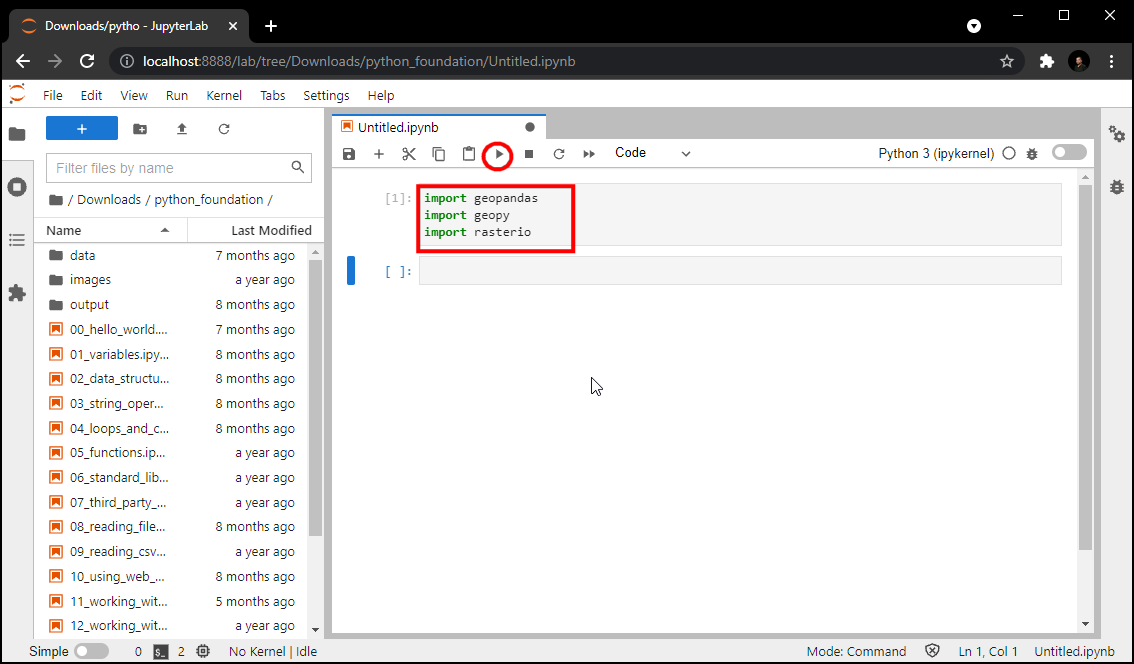使用 Jupyter Notebooks

1. 运行JupyterLab 程序，会打开你的默认浏览器，加载一个新的标签页。从左边的面板中，找到本课程所提供压缩包解压后的位置。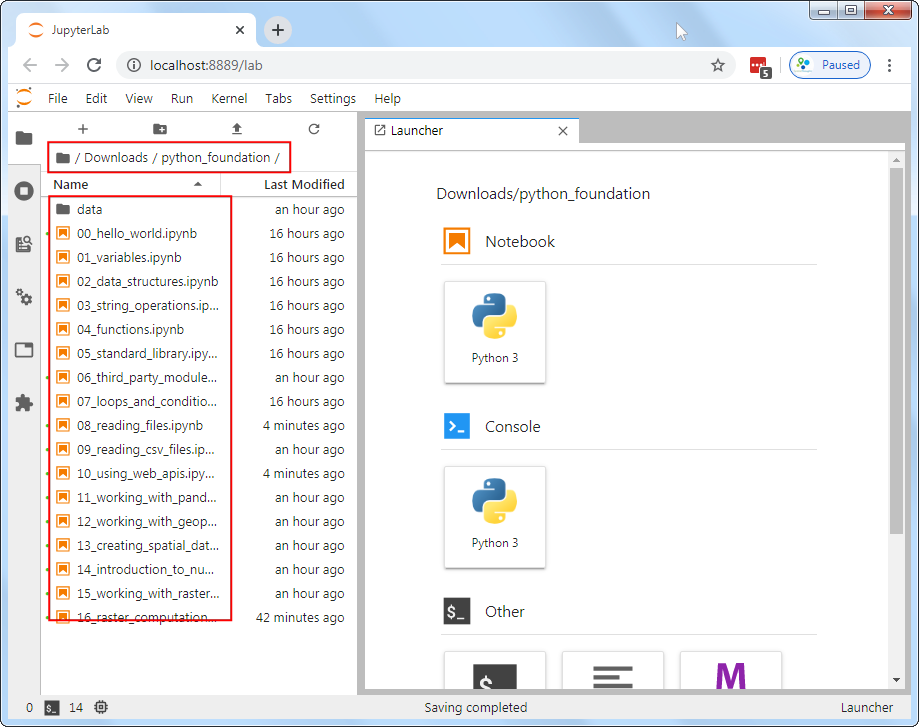1. Jupyter notebooks 的文件后缀是.ipynb。在左侧的面板中找到一个这样的文件双击来打开。这里面的代码是一个个按照块（cell）来执行的。你可以选择一个块，然后点击运行按钮Run来运行一下看看输出是什么样的。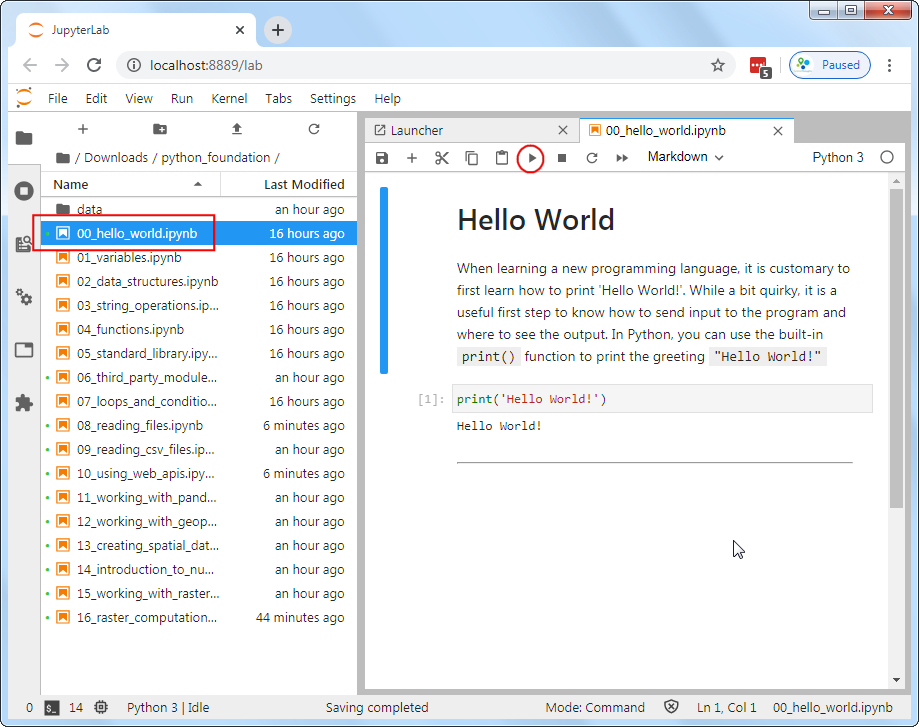1. 每个notebook结尾，都有一个对应的联系。在添加新代码块来完成练习之前，一定要记得到Run → Run All Cells来执行完毕整个 notebook 中的所有代码。这会保证你需要在练习中用到的变量都可用。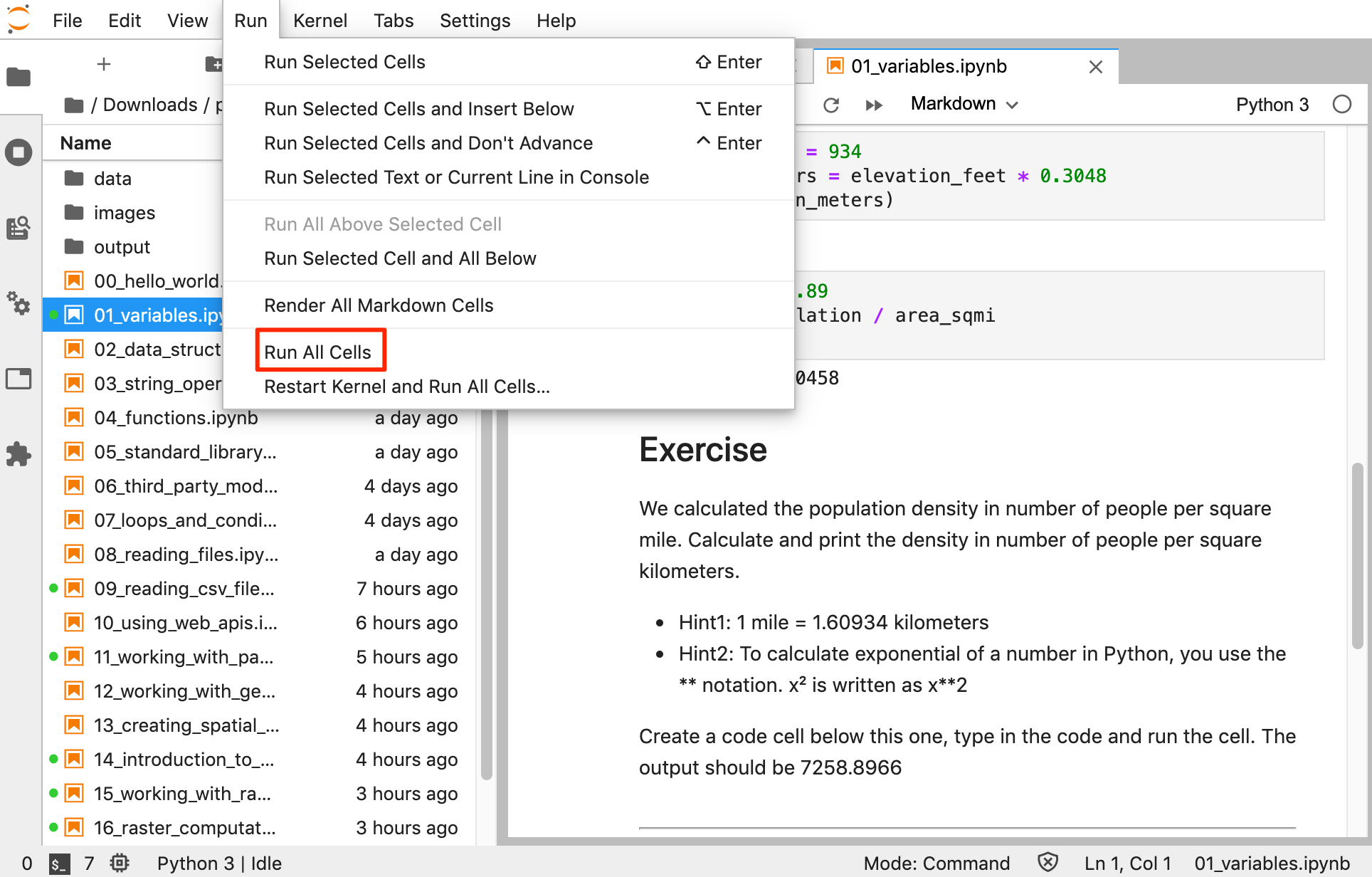Hello World

print('Hello World!')

变量类型

字符串（Strings）

Python 里面使用单引号或者双引号来创建字符串。

city = 'San Fransico'
state = 'California'
print(city, state)
print(city + state)
print(city + ',' + state)

数值（Numbers）

Python支持的数值类型很多，不过最常用的就是下面这两种：

• int，整数
• float，浮点数
population = 881549
latitude = 37.7739
longitude = -121.5687
print(type(population))
print(type(latitude))
elevation_feet = 934
elevation_meters = elevation_feet * 0.3048
print(elevation_meters)
area_sqmi = 46.89
density = population / area_sqmi
print(density)

练习：单位转换

• 提示1: 1 mile 英里 = 1.60934 kilometers 公里

distance_km = 4135
# Remove this line and add code here

数据结构

元组（Tuples）

latitude = 37.7739
longitude = -121.5687
coordinates = (latitude, longitude)
print(coordinates)

y = coordinates
x = coordinates
print(x, y)

列表（Lists）

cities = ['San Francisco', 'Los Angeles', 'New York', 'Atlanta']
print(cities)

print(cities)

print(len(cities))

cities.append('Boston')
print(cities)

print(len(cities))

cities.sort()
print(cities)

cities.sort(reverse=True)
print(cities)

集合（Sets）

capitals = ['Sacramento', 'Boston', 'Austin', 'Atlanta']
capitals_set = set(capitals)
cities_set = set(cities)

capital_cities = capitals_set.intersection(cities_set)
print(capital_cities)

cities.extend(capitals)
print(cities)
print(set(cities))

字典（Dictionaries）

Python中的字典是用大括号{}括起来的。字典由键名keys和值values组成的键值对构成。列表和元组是按照顺序来读取元素，而字典则是要通过键名来读取元素。键和值之间用冒号:分隔。

data = {'city': 'San Francisco', 'population': 881549, 'coordinates': (-122.4194, 37.7749) }
print(data)

print(data['city'])

练习

40.661
-73.944
nyc_data = {'city': 'New York', 'population': 8175133, 'coordinates': (40.661, -73.944) }

字符运算（String Operations）

city = 'San Francisco'
print(len(city))
print(city.split())
print(city.upper())
city
city[-1]
city[0:3]
city[4:]

转义字符（Escaping characters）

Python语言本身有很多特殊字符有特定用途。比如单引号 就用来定义字符串。如果你就想要一个字符串包含这样一个单引号该怎么办？

# my_string = 'It's a beautiful day!'

my_string = 'It\'s a beautiful day!'
print(my_string)

my_string = "It's a beautiful day!"

latitude = '''37° 46' 26.2992" N'''
longitude = '''122° 25' 52.6692" W'''
print(latitude, longitude)

#path = 'C:\Users\ujaval'
#print(path)

path = r'C:\Users\ujaval'
print(path)

打印字符串（Printing Strings）

city = 'San Fransico'
population = 881549
output = 'Population of {} is {}.'.format(city, population)
print(output)

latitude = 37.7749
longitude = -122.4194

coordinates = '{:.2f},{:.2f}'.format(latitude, longitude)
print(coordinates)

练习

37
46
26.2992
latitude = '''37° 46' 26.2992"'''

循环和条件

For 循环

For 循环要使用一个序列进行迭代（iterating）。这个序列可以是列表、元组、字典、集合，或者字符串。

cities = ['San Francisco', 'Los Angeles', 'New York', 'Atlanta']

for city in cities:
print(city)

data = {'city': 'San Francisco', 'population': 881549, 'coordinates': (-122.4194, 37.7749) }

for x, y in data.items():
print(x, y)

for x in range(5):
print(x)

for x in range(1, 10, 2):
print(x)

条件判断

Python支持的逻辑条件包括等于、不等、大于、小于、大于等于、小于等于等等。这些条件可以有多种用法，最常见的是放在if语句和循环体中。

for city in cities:
if city == 'Atlanta':
print(city)

if配合使用的else就可以做多个条件的判断了。

for city in cities:
if city == 'Atlanta':
print(city)
else:
print('This is not Atlanta')

Python的代码要靠缩进来区分段落。所以一定要做好代码缩进。 使用elif还可以评估一系列的判断条件。 多个条件的组合可以使用andor

cities_population = {
'San Francisco': 881549,
'Los Angeles': 3792621,
'New York': 8175133,
'Atlanta':498044
}

for city, population in cities_population.items():
if population < 1000000:
print('{} is a small city'.format(city))
elif population > 1000000 and population < 5000000:
print('{} is a big city'.format(city))
else:
print('{} is a mega city'.format(city))

控制语句

For循环每次在序列中迭代一个元素。有时候可能要停止执行，或者跳过某部分。这时候可以使用breakcontinuepass这三种语句。

break 停止循环并跳出。

for city in cities:
print(city)
if city == 'Los Angeles':
print('I found Los Angeles')
break

continue 跳过循环体内剩余部分直接进行下一部分迭代。

for city in cities:
if city == 'Los Angeles':
continue
print(city)

pass 语句什么都不做。这个在你需完成一些语法结构但还不希望任何代码执行的时候就很有用。一般用作未完成函数部分的占位符。

for city in cities:
if city == 'Los Angeles':
pass
else:
print(city)

练习

Fizz Buzz挑战。

1, 2, Fizz, 4, Buzz, Fizz, 7, 8, Fizz, Buzz, 11, Fizz, 13, 14, FizzBuzz, ...

• 如果一个数同时是3和5的倍数，输出FizzBuzz
• 如果一个数是3的倍数，输出Fizz
• 如果一个数是5的倍数，输出Buzz
• 其他情况输出这个数本身

for x in range(1, 10):
if x%2 == 0:
print('{} is divisible by 2'.format(x))
else:
print('{} is not divisible by 2'.format(x))

函数（Functions）

def my_function():
....
....
return something

def greet(name):
return 'Hello ' + name

print(greet('World'))

• 1 度（degree） 等于 60 分钟（minutes）
• 1 分（minute）等于 60 秒（seconds）

def dms_to_decimal(degrees, minutes, seconds):
if degrees < 0:
result = degrees - minutes/60 - seconds/3600
else:
result = degrees + minutes/60 + seconds/3600
return result

output = dms_to_decimal(10, 10, 10)
print(output)

练习

def dms_to_decimal(degrees, minutes, seconds):
if degrees < 0:
result = degrees - minutes/60 - seconds/3600
else:
result = degrees + minutes/60 + seconds/3600
return result

coordinate = '''37° 46' 26.2992"'''

# 在下面添加代码，从字符串中提取出坐标数值
# 然后调用函数将坐标转换成十进制度并打印输出
# 正确结果应该是 37.773972
# 提示：先将字符串转换成淑芝
# 当你直接从字符串中提取坐标的时候，提取到的是字符串
# 所以你需要使用内置的浮点数转换函数foat()来将其转换成数值
x = '25'
print(x, type(x))
y = int(x)
print(y, type(y))

Python 标准库（Standard Library）

Python 有内置的很多模块，提供了很多方便的功能，能解决各种常见变成问题。要使用这些模块，你必须用import来导入。导入到你的Python代码之后，就可以在你的代码中使用这个模块提供的函数了。

import math

from math import pi
print(pi)

计算距离

san_francisco = (37.7749, -122.4194)
new_york = (40.661, -73.944)
def haversine_distance(origin, destination):
lat1, lon1 = origin
lat2, lon2 = destination
a = math.sin(dlat/2) * math.sin(dlat/2) + math.cos(math.radians(lat1)) \
* math.cos(math.radians(lat2)) * math.sin(dlon/2) * math.sin(dlon/2)
c = 2 * math.atan2(math.sqrt(a), math.sqrt(1-a))
return distance
distance = haversine_distance(san_francisco, new_york)
print(distance/1000, 'km')

探索 Python 的彩蛋

import this

Let’s try one more. Try importing the antigravity module.

练习

def haversine_distance(origin, destination):
lat1, lon1 = origin
lat2, lon2 = destination
a = math.sin(dlat/2) * math.sin(dlat/2) + math.cos(math.radians(lat1)) \
* math.cos(math.radians(lat2)) * math.sin(dlon/2) * math.sin(dlon/2)
c = 2 * math.atan2(math.sqrt(a), math.sqrt(1-a))
return distance

# city1 = (lat1, lng1)
# city2 = (lat2, lng2)
# call the function and print the result

第三方模块

Python的第三方模块（也可以叫做库或者包）有一个非常繁荣的生态。有成千上万个第三方模块，你可以安装并使用。

安装第三方模块

Python的包管理器是pip。所有可安装的包都可以在 PyPI (Python Package Index)中找到。在终端中运行下面的命令就可以安装一个包了：

pip install <package name>

conda install <package name>

计算距离

• distance.great_circle(): 使用哈维辛方程（haversine formula）计算大圆距离
• distance.geodesic(): 使用文森特方程（vincenty’s formula）计算所选的椭球提距离
from geopy import distance

san_francisco = (37.7749, -122.4194)
new_york = (40.661, -73.944)

straight_line_distance = distance.great_circle(san_francisco, new_york)
ellipsoid_distance = distance.geodesic(san_francisco, new_york, ellipsoid='WGS-84')

print(straight_line_distance, ellipsoid_distance)

练习

from geopy import distance

# city1 = (lat1, lng1)
# city2 = (lat2, lng2)
# call the geopy distance function and print the great circle and ellipsoid distance

使用网络接口（API）

API 是 Application Program Interface 的缩写，意思是应用程序接口，允许一个程序和另一个程序进行沟通。很多网站或者服务商都提供了API，允许以自动的方式查询信息。

• 获取方向、导航
• 路径最优化
• 地理位置编码（Geocoding）
• 下载数据
• 获取实时天气数据

理解 JSON 和 GeoJSON

JSON 是 JavaScript Object Notation 的缩写。这个文件格式是用来存储和传输数据的，也是接口之间进行数据传输的事实上的标准。GeoJSON就是对JSON格式的扩展，使之用于表征空间数据。

Python 有一个内置的json模块，内部的方法可以读取JSON并将其转化成Python对象，还可以反过来将Python对象存储成JSON文件。在这个例子中，我们使用requests 模块来查询接口来实现转化。Python中最基础的JSON方法也有必要学习一下。

GeoJSON 数据包含很多特征（features），其中每个特征都有若干属性（properties）和集合信息（geometry）。

geojson_string = '''
{
"type": "FeatureCollection",
"features": [
{"type": "Feature",
"properties": {"name": "San Francisco"},
"geometry": {"type": "Point", "coordinates": [-121.5687, 37.7739]}
}
]
}
'''
print(geojson_string)

import json

print(type(data))
print(data)

city_data = data['features']
print(city_data)

city_name = city_data['properties']['name']
city_coordinates = city_data['geometry']['coordinates']
print(city_name, city_coordinates)

requests模块

import requests

response = requests.get('https://www.spatialthoughts.com')

print(response.status_code)

使用 OpenRouteService API 计算两点间距离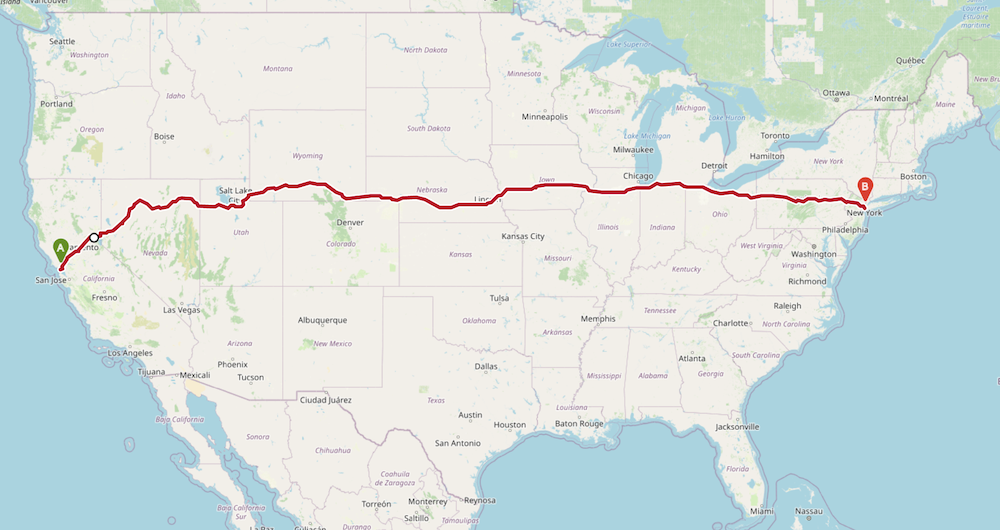OpenRouteService (ORS) 提供了免费接口，可以用于路径规划和导航的、距离矩阵、地理编码、路径最优化等等。接下来我们既要学习通过Python来使用此接口，来获取现实世界中两个城市之间的距离。

ORS_API_KEY = '<replace this with your key>'

import requests

san_francisco = (37.7749, -122.4194)
new_york = (40.661, -73.944)

parameters = {
'api_key': ORS_API_KEY,
'start' : '{},{}'.format(san_francisco, san_francisco),
'end' : '{},{}'.format(new_york, new_york)
}

response = requests.get(
'https://api.openrouteservice.org/v2/directions/driving-car', params=parameters)

if response.status_code == 200:
print('Request successful.')
data = response.json()
else:
print('Request failed.')

data = response.json()

summary = data['features']['properties']['summary']
print(summary)

distance = summary['distance']
print(distance/1000)

接口频率限制（API Rate Limiting）

OpenRouteService 列出了几条接口限制API Restrictions。免费用户最多允许每分钟40次导航请求。

time模块

Python标准库（Standard Library）内置了一个time模块，可以进行各种时间相关的运算。其中的一个方法time.sleep()可以在指定的时间内暂停程序执行。

import time
for x in range(10):
print(x)
time.sleep(1)

练习

import csv
import os
import requests
import time
ORS_API_KEY = '<replace this with your key>'

def get_driving_distance(source_coordinates, dest_coordinates):
parameters = {
'api_key': ORS_API_KEY,
'start' : '{},{}'.format(source_coordinates, source_coordinates),
'end' : '{},{}'.format(dest_coordinates, dest_coordinates)
}

response = requests.get(
'https://api.openrouteservice.org/v2/directions/driving-car', params=parameters)

if response.status_code == 200:
data = response.json()
summary = data['features']['properties']['summary']
distance = summary['distance']
return distance/1000
else:
print('Request failed.')
return -9999

san_francisco = (37.7749, -122.4194)

destination_cities = {
'Los Angeles': (34.0522, -118.2437),
'Boston': (42.3601, -71.0589),
'Atlanta': (33.7490, -84.3880)
}

Python提供了内置函数进行文件读写。 想要读取一个文件，就必须要知道它存储在磁盘的路径。Python内置一个 os 模块可以帮助解决操作系统方面的问题。使用 os 的一个好处就是你写的代码不用修改就可以在其支持的操作系统上运行。

import os

data_pkg_path = 'data'
filename = 'worldcities.csv'
path = os.path.join(data_pkg_path, filename)
print(path)

f = open(path, 'r', encoding='utf-8')
f.close()

f = open(path, 'r', encoding='utf-8')

count = 0
for line in f:
count += 1
f.close()
print(count)

练习

• 提示：使用 break 语句
import os
data_pkg_path = 'data'
filename = 'worldcities.csv'
path = os.path.join(data_pkg_path, filename)

# 在此处添加代码来读取文件的前五行

读取CSV（逗号分隔符）文件

CSV 是 Comma-separated Value 的缩写，意思是逗号分隔符，是一种很常用的文本文件格式，可以用于存储地理空间信息。文件中每行中的各个列（columns）都通过一个英文字符逗号（comma）来分隔开。（译者注：新手常犯的错误就是混用中英文标点符号，一定要记得CSV当中用的是英文字符的逗号，不是中文输入法下的中文逗号。实际上，推荐大家尽量在写代码的过程中一律使用英文字符的标点符号，这样能减少很多麻烦。）

import csv

import os
data_pkg_path = 'data'
filename = 'worldcities.csv'
path = os.path.join(data_pkg_path, filename)
f = open(path, 'r')
f.close()

使用枚举函数enumerate()

cities = ['San Francisco', 'Los Angeles', 'New York', 'Atlanta']
for x in enumerate(cities):
print(x)

f = open(path, 'r', encoding='utf-8')
print(row)
if index == 4:
break
f.close()

使用 with 语句

with open(path, 'r', encoding='utf-8') as f:

筛选行

home_country = 'Italy'
num_cities = 0

with open(path, 'r', encoding='utf-8') as f:

if row['country'] == home_country:
num_cities += 1

print(num_cities)

计算距离

home_city = 'Rome'

home_city_coordinates = ()

with open(path, 'r', encoding='utf-8') as f:
if row['city_ascii'] == home_city:
lat = row['lat']
lng = row['lng']
home_city_coordinates = (lat, lng)
break

print(home_city_coordinates)

from geopy import distance

counter = 0
with open(path, 'r', encoding='utf-8') as f:
if (row['country'] == home_country and
row['city_ascii'] != home_city):
city_coordinates = (row['lat'], row['lng'])
city_distance = distance.geodesic(
city_coordinates, home_city_coordinates).km
print(row['city_ascii'], city_distance)
counter += 1

if counter == 5:
break

写入文件

output_dir = 'output'
if not os.path.exists(output_dir):
os.mkdir(output_dir)
output_filename = 'cities_distance.csv'
output_path = os.path.join(output_dir, output_filename)

with open(output_path, mode='w', encoding='utf-8') as output_file:
fieldnames = ['city', 'distance_from_home']
csv_writer = csv.DictWriter(output_file, fieldnames=fieldnames)

# Now we read the input file, calculate distance and
# write a row to the output
with open(path, 'r', encoding='utf-8') as f:
if (row['country'] == home_country and
row['city_ascii'] != home_city):
city_coordinates = (row['lat'], row['lng'])
city_distance = distance.geodesic(
city_coordinates, home_city_coordinates).km
csv_writer.writerow(
{'city': row['city_ascii'],
'distance_from_home': city_distance}
)

import csv
import os
from geopy import distance

data_pkg_path = 'data'
input_filename = 'worldcities.csv'
input_path = os.path.join(data_pkg_path, input_filename)
output_filename = 'cities_distance.csv'
output_dir = 'output'
output_path = os.path.join(output_dir, output_filename)

if not os.path.exists(output_dir):
os.mkdir(output_dir)

home_city = 'Bengaluru'
home_country = 'India'

with open(input_path, 'r', encoding='utf-8') as input_file:
if row['city_ascii'] == home_city:
home_city_coordinates = (row['lat'], row['lng'])
break

with open(output_path, mode='w') as output_file:
fieldnames = ['city', 'distance_from_home']
csv_writer = csv.DictWriter(output_file, fieldnames=fieldnames)

with open(input_path, 'r', encoding='utf-8') as input_file:
if (row['country'] == home_country and
row['city_ascii'] != home_city):
city_coordinates = (row['lat'], row['lng'])
city_distance = distance.geodesic(
city_coordinates, home_city_coordinates).km
csv_writer.writerow(
{'city': row['city_ascii'],
'distance_from_home': city_distance}
)

print('Successfully written output file at {}'.format(output_path))

练习

home_cityhome_country变量设成某个城市和国家地区，然后创建一个某国家或地区的某城市到其他城市距离列表的CSV文件。

使用PandasPandas 是一个非常强大的数据处理库，提供了简便高效的函数来读取和分析文件中的数据。

Pandas 基于另一个库numpy，也广泛用于科学计算。Pandas扩展了numpy，提供了新的数据类型，比如IndexSeriesDataFrames

Pandas的实现非常快速高效，和其他数据处理方法相比，使用pandas能够让代码更简单快速。接下来将上面的文件读取和距离计算的代码用Pandas重新实现一下。

import pandas as pd

读取文件

import os
data_pkg_path = 'data'
filename = 'worldcities.csv'
path = os.path.join(data_pkg_path, filename)

print(df.info())

筛选数据

Pandas有很多从一个DataFrame来选择和筛选数据的方法。接下来试试如何使用布尔筛选 Boolean Filtering来筛选出满足条件的行。

filtered = df[df['country'] == home_country]
print(filtered)

country_df = df[df['country'] == home_country].copy()

home_city = 'Rome'
filtered = country_df[country_df['city_ascii'] == home_city]
print(filtered.iloc)

home_city_coordinates = (filtered.iloc['lat'], filtered.iloc['lng'])
print(home_city_coordinates)

运行计算

apply()函数接收两个参数。一个是要应用的函数，另一个是对应的轴方向。比如axis=0意思是对每一列运行，而axis=1则表示应用到各行。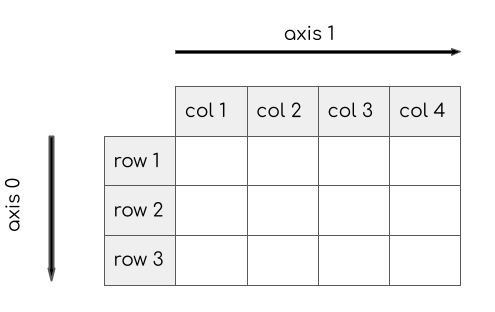from geopy import distance

def calculate_distance(row):
city_coordinates = (row['lat'], row['lng'])
return distance.geodesic(city_coordinates, home_city_coordinates).km

result = country_df.apply(calculate_distance, axis=1)
print(result)

country_df['distance'] = result
print(country_df)

filtered = country_df[['city_ascii','distance']]
print(filtered)

city_ascii 列重命名一个更可读的名字。

filtered = filtered.rename(columns = {'city_ascii': 'city'})
print(filtered)

output_filename = 'cities_distance_pandas.csv'
output_dir = 'output'
output_path = os.path.join(output_dir, output_filename)
filtered.to_csv(output_path, index=False)
print('Successfully written output file at {}'.format(output_path))

使用 GeopandasGeoPandas扩展了Pandas库，增加了空间分析操作，还提供了新的数据类型，比如GeoDataFrameGeoSeries分别是DataFrameSeries的子类 ，允许用Python进行向量数据的高效处理。

GeoPandas使用了很多其他广泛应用的空间分析库，但提供了和Pandas相似的洁面，因此很容易用来进行空间分析。GeoPandas基于下面这些库。

• Shapely用于几何运算（缓冲、交叉等等）；
• PyProj 处理投影；
• Fiona 用于文件输入输出，而本身又基于GDAL/OGR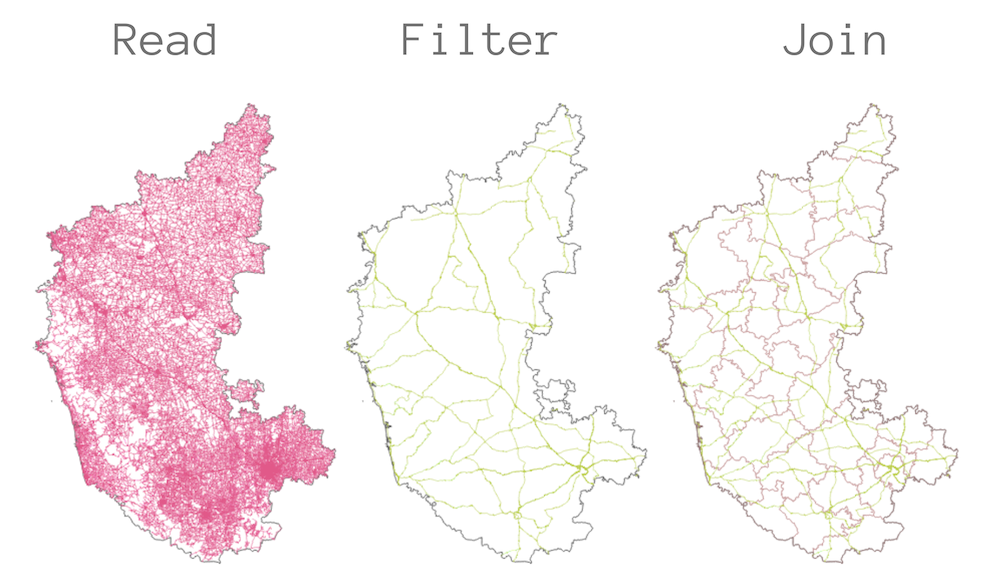import geopandas as gpd

读取空间数据

import os
data_pkg_path = 'data'
filename = 'karnataka.gpkg'
path = os.path.join(data_pkg_path, filename)

处理投影

print(filtered.crs)

print('Total length of national highways in the state is {} KM'.format(total_length/1000))

进行空间连接

districts_reprojected = districts_gdf.to_crs('EPSG:32643')

• op: 空间动作（spatial predicate），决定要连接的对象。选项包括intersectswithincontains（交叉、在内、包含）。
• how: 连接的类型。选项包括leftrightinner（左、右、内）。

joined = gpd.sjoin(roads_reprojected, districts_reprojected, how='left', op='intersects')

分组统计（Group Statistics）

results = joined.groupby('DISTRICT')['length'].sum()/1000
print(results)

group_by()的方法得到的结果是一个Pandas的系列Series数据类型。可以使用to_csv()方法将其存储成CSV文件。

output_filename = 'national_highways_by_districts.csv'
output_dir = 'output'
output_path = os.path.join(output_dir, output_filename)
results.to_csv(output_path)
print('Successfully written output file at {}'.format(output_path))

创建空间数据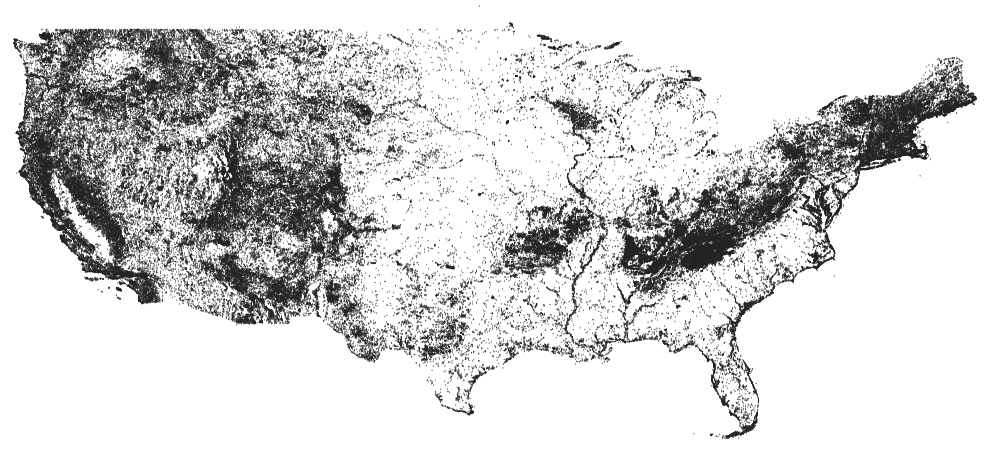import os
import pandas as pd
import geopandas as gpd
data_pkg_path = 'data/geonames/'
filename = 'US.txt'
path = os.path.join(data_pkg_path, filename)

读取制表符分隔文件（Tab-Delimited Files）

column_names = [
'geonameid', 'name', 'asciiname', 'alternatenames',
'latitude', 'longitude', 'feature class', 'feature code',
'dem', 'timezone', 'modification date'
]

print(df.info())

筛选数据

mountains = df[df['feature class']=='T']

创建几何体

GeoPandas有一个很方便的points_from_xy()函数，可以使用X和Y坐标创建一个几何体列。由此可以接收一个Pandas的DataFrame，然后创建一个GeoDataFrame，带有指定的坐标参考系（CRS）和几何体列。

geometry = gpd.points_from_xy(mountains.longitude, mountains.latitude)
gdf = gpd.GeoDataFrame(mountains, crs='EPSG:4326', geometry=geometry)
print(gdf.info())

写入文件

output_dir = 'output'
output_filename = 'mountains.gpkg'
output_path = os.path.join(output_dir, output_filename)

gdf.to_file(driver='GPKG', filename=output_path, encoding='utf-8')
print('Successfully written output file at {}'.format(output_path))

练习

• 提示1: 使用os.listdir()方法来获得一个路径中的所有文件。
• 提示2: 使用Pandas的方法concat()来合并多个DataFrame。
import os
import pandas as pd
import geopandas as gpd

data_pkg_path = 'data/geonames/'
files = os.listdir(data_pkg_path)

filepaths = []
for file in files:
filepaths.append(os.path.join(data_pkg_path, file))
print(filepaths)

# 迭代所有文件，使用Pandas读取然后创建DataFrame列表。
# 然后使用pd.concat()函数进行合并

NumPy 简介NumPy (Numerical Python)是一个用于科学计算的重要的Python库。Pandas和GeoPandas这样的库都基于NumPy。

NumPy提供了处理数组Arrays的快速高效方式。在空间数据分析领域中，NumPy在处理卫星图像、航拍照片、高程数据等栅格数据（Raster data）的时候发挥了重要作用。由于这些栅格数据是每个通道二维数组的结构，所以学习NumPy对于使用Python来处理栅格数据来说也是至关重要的。

import numpy as np

数组（Arrays）

NumPy的数据对象叫做ndarray。这一数据类型提供了很多支持函数，处理数组又快又方便。数组（Array）和Python的列表很相似，但ndarray在数学运算上至少要更快50倍。可以使用array()方法来创建一个数组。得到的对象就是numpy.ndarray

a = np.array([1, 2, 3, 4])
print(type(a))

b = np.array([[1, 2, 4], [3, 4, 5]])
print(b)
print(b.ndim)
print(b.shape)

print(b)
print(b)

数组运算（Array Operations）

NumPy数组上的数学运算方便又快捷。NumPy有很多内置函数可以用于常见运算。

print(np.sum(b))

c = np.array([[2, 2, 2], [2, 2, 2]])
print(np.divide(b, c))

print(b/c)

print(b)
print(b*2)
print(b/2)

NumPy的一个重要概念就是数组轴（Array Axes）。和pandas库类似，在二维数组中，0轴是行方向，1轴是列方向。下图展示的就是数组轴方向。print(b)
row_sum = b.sum(axis=0)
print(row_sum)

练习

import numpy as np

b = np.array([[1, 2, 4], [3, 4, 5]])
print(b)

使用 RasterIO

RasterIO是一个用于处理网格格式的地理空间数据的第三方库。该库提供了一个用于读写栅格数据的简单方式，也可以读取各波段数据，将像素作为numpy数组。

RasterIO基于流行的地理空间数据抽象库 GDAL (Geospatial Data Abstraction Library)。GDAL是用C++写的，所以其提供的Python接口对Python用户来说挺难适应。RasterIO的目的是让Python用户以更符合直觉的方式来使用GDAL库。import rasterio
import os
data_pkg_path = 'data'
srtm_dir = 'srtm'
filename = 'N28E087.hgt'
path = os.path.join(data_pkg_path, srtm_dir, filename)

读取栅格数据

RasterIO可以读取任何GDAL支持的栅格数据文件。可以对栅格数据文件路径调用open()函数来打开。得到的数据集就像是一个Python里面的文件对象。

dataset = rasterio.open(path)

print(band1)

dataset.close()

合并数据集

srtm_path = os.path.join(data_pkg_path, 'srtm')
all_files = os.listdir(srtm_path)
print(all_files)

rasterio.merge模块有一个merge()方法，接收一个数据集列表，返回合并的数据集。所以茶u你国军爱你一个空列表，然后打开每个文件，将其添加到列表中。

dataset_list = []
for file in all_files:
path = os.path.join(srtm_path, file)
dataset_list.append(rasterio.open(path))
print(dataset_list)

from rasterio import merge
merged_result = merge.merge(dataset_list)
print(merged_result)

merged_data = merged_result
merged_transform = merged_result

print(merged_data.shape)

写入栅格数据

output_filename = 'merged.tif'
output_dir = 'output'
output_path = os.path.join(output_dir, output_filename)

new_dataset = rasterio.open(output_path, 'w',
driver='GTiff',
height=merged_data.shape,
width=merged_data.shape,
count=1,
nodata=-32768.0,
dtype=merged_data.dtype,
crs='+proj=latlong',
transform=merged_transform)
new_dataset.write(merged_data)
new_dataset.close()
print('Successfully written output file at {}'.format(output_path))

练习

import rasterio
import os
import numpy as np

output_filename = 'merged.tif'
output_dir = 'output'
output_path = os.path.join(output_dir, output_filename)

# 将输出文件读取为NumPy数组然后找到最大值

写独立的Python脚本

写一个脚本

from geopy import distance

san_francisco = (37.7749, -122.4194)
new_york = (40.661, -73.944)

ellipsoid_distance = distance.geodesic(san_francisco, new_york, ellipsoid='WGS-84').km

print('Source Coordinates: {},{}'.format(san_francisco, san_francisco))
print('Destination Coordinates: {},{}'.format(san_francisco, san_francisco))
print('Elliosoid Distance: {} km'.format(ellipsoid_distance))

运行脚本

Windows

1. 运行 Anaconda Navigator 程序。选择python_foundation环境，然后运行CMD.exe Prompt程序。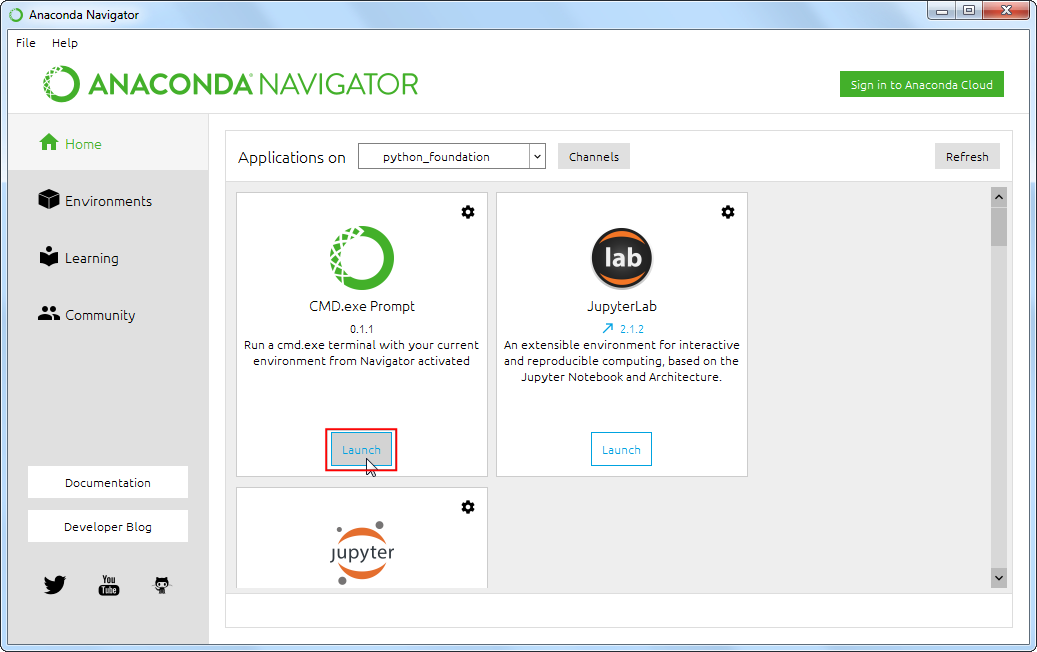1. 定位到包含脚本的文件夹，使用cd命令。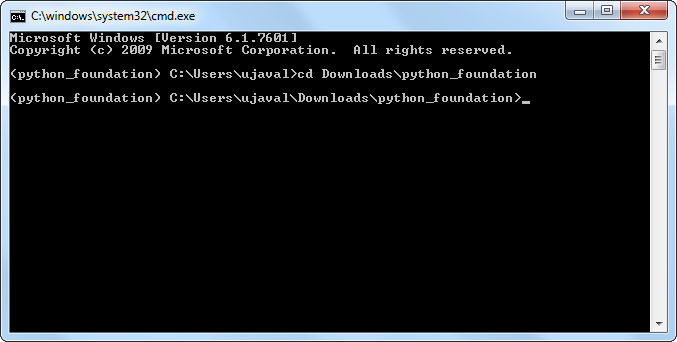1. 使用python命令来运行脚本，脚本会输出计算出的距离。
python get_distance.py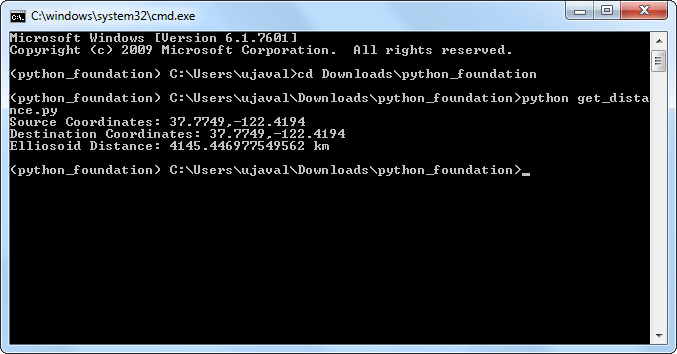macOS 和 Linux

1. 打开终端（Terminal）。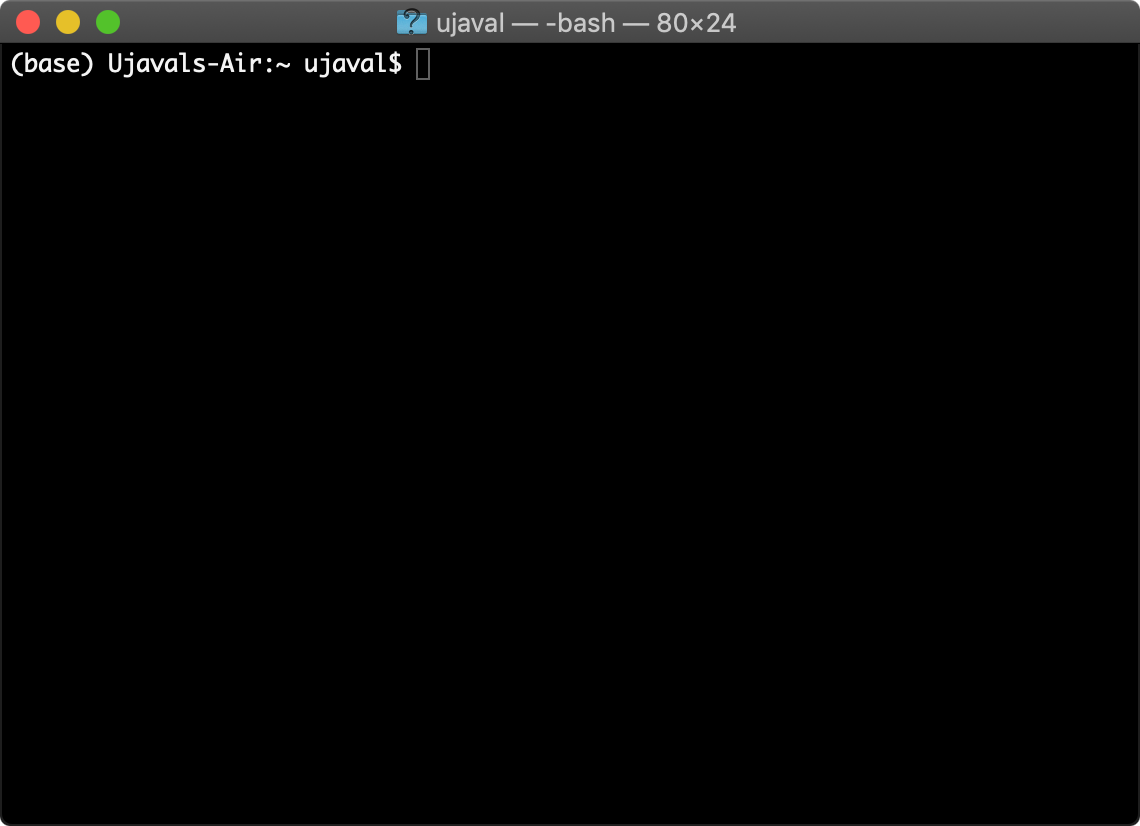1. 切换到当前的conda环境。
conda activate python_foundation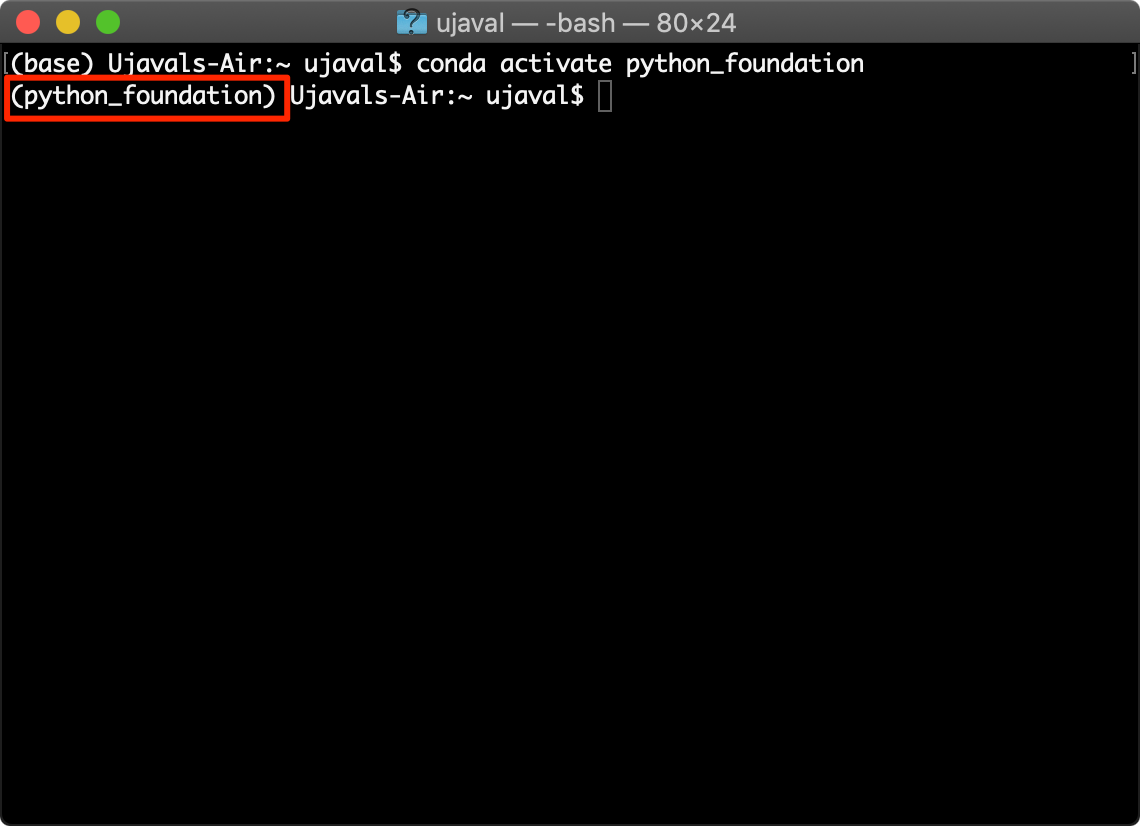1. 定位到包含脚本的文件夹，使用cd命令。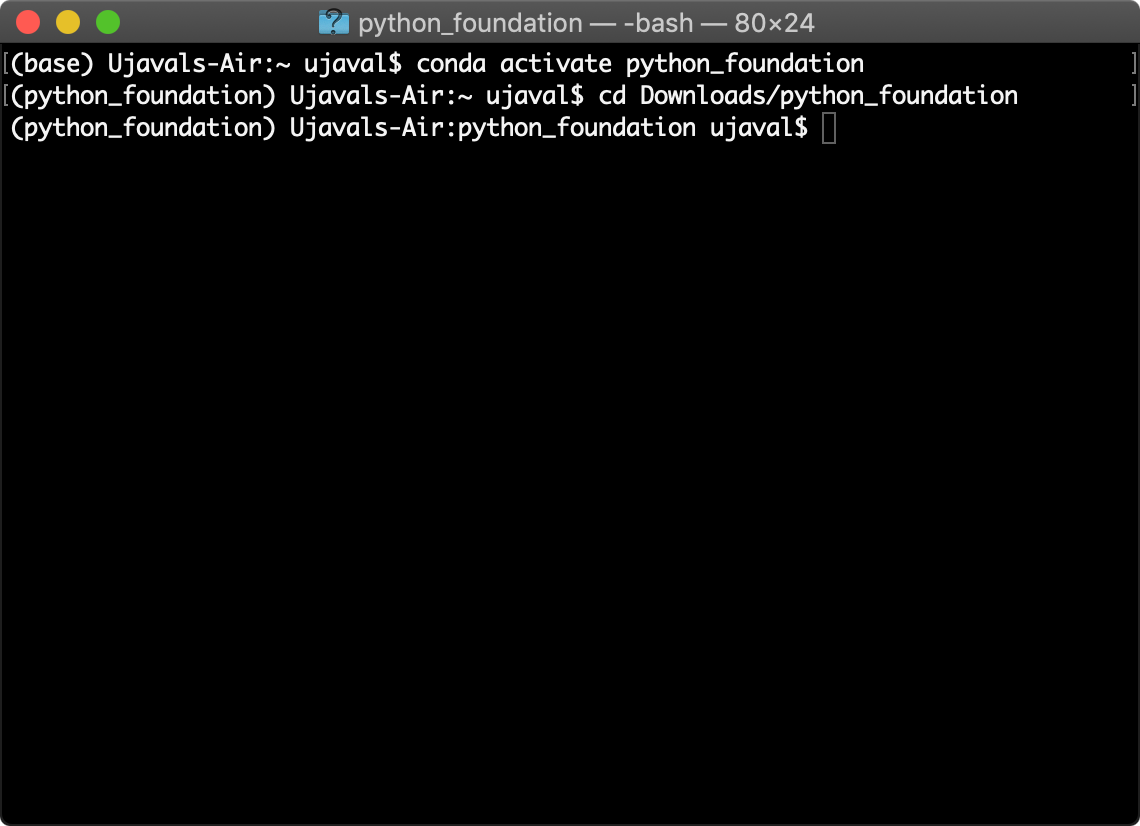1. 使用python命令来运行脚本，脚本会输出计算出的距离。

如果你的系统安装了多个Python，就需要选择正确的Python实例。

python get_distance.py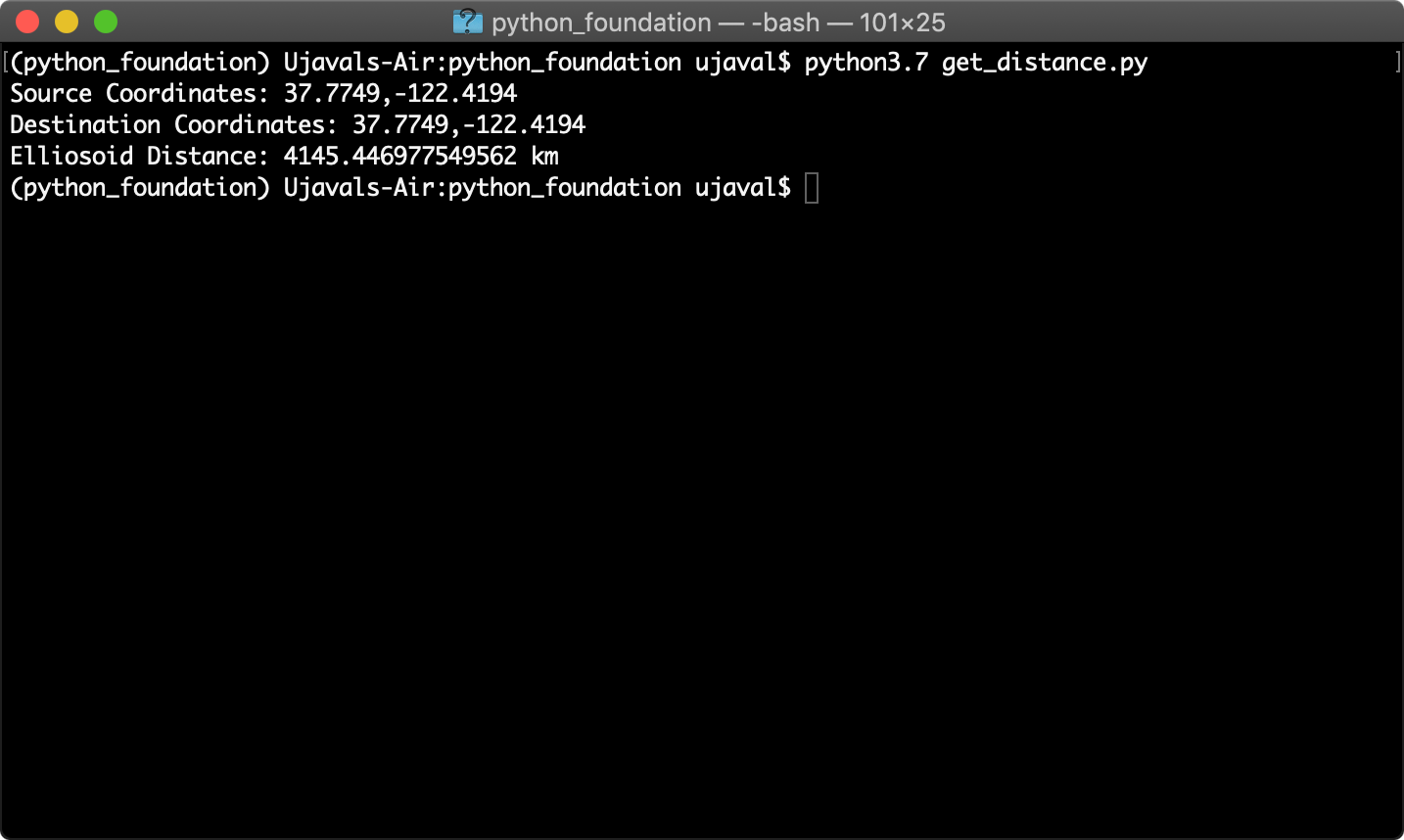接下来呢？

做项目

• 地理信息编码 Geocoding: 找一系列的地址列表，然后对其进行地理信息编码，创建一个点图层，可以参考 geocoding using geopy
• 网络分析 Network Analysis: 使用osmnx 包来利用OpenStreetMap数据使用Python进行网络分析。开始以参考此处的例子
• 可视化和交互地图: 使用投图库geoplot或者地图绘制库folium 来创建一个交互地图。可以参考此处的例子
• 地理信息处理 Geo-processing: 之前有若干中级水平的地理信息处理的简介，使用的是QGIS。可以参考下面链接中的教程，然后用geopandasrasterio这两个库来实现。

补充信息

使用GeoPandas创建地图

GeoPandas有一些内置的函数可以对地理空间数据进行可视化以及建立地图。实际上使用的是强大的matplotlib库来进行投图的。如果你对matplotlib不太熟悉，可以参考相关导论

import geopandas as gpd
import os
data_pkg_path = 'data'
filename = 'karnataka.gpkg'
path = os.path.join(data_pkg_path, filename)

Matplotlib 基础

%matplotlib inline
import matplotlib.pyplot as plt

• Figure: 这就是投图plot的主容器。一个Figure可以包含多个Plot。
• Axes: Axes表示的是一个独立的Plot或者图（Graph），一个Figure可以包含1个或多个Axes。

渲染地图输出

subplots()函数在一个figure内创建一个或多个plots。可以设计一个多行多列的地图。在下面的代码中，创建的是一行三列的地图。使用了set_size_inches()函数将地图的规格设置为15 x 7英寸。

fig, axes = plt.subplots(1, 3)
fig.set_size_inches(15,7)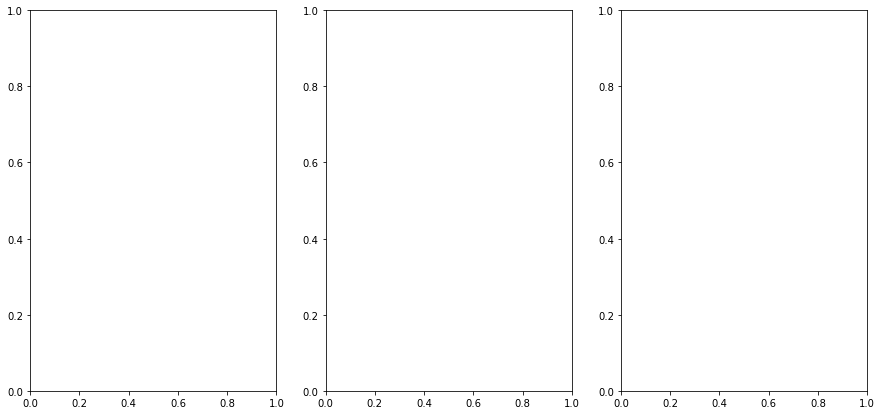subplots() 返回两个项目。一个是图，另一个是figure内所有axes组成的远足。由于我们有三个axes，就将它们解包获得分开的几个变量。

ax0, ax1, ax2 = axes

GeoDataFrame对象有一个plot()方法，使用的是pyplot来创建投图。这里将ax对象传递给函数，然后得到的投图结果就会显示在前面创建的Axes上来。这里添加districts多边形图层到ax0对象中，也就是第一个subplot。

districts.plot(ax=ax0, linewidth=1, facecolor='none', edgecolor='#252525')
fig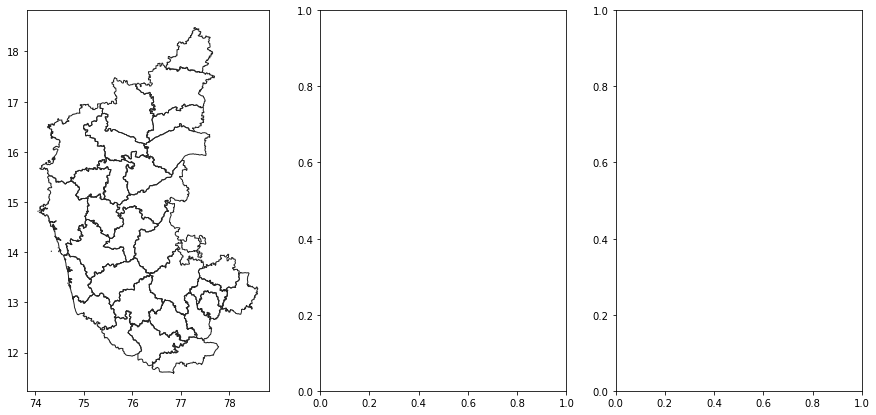<Figure size 432x288 with 0 Axes>

fig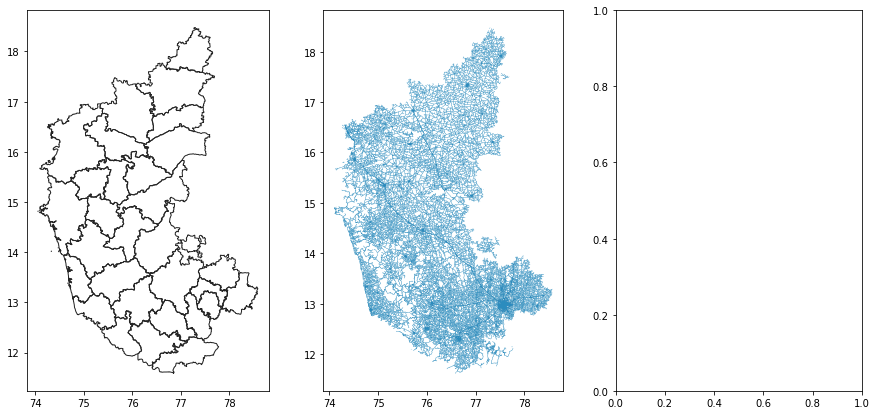<Figure size 432x288 with 0 Axes>

national_highways.plot(ax=ax2, linewidth=1, color='#de2d26')
fig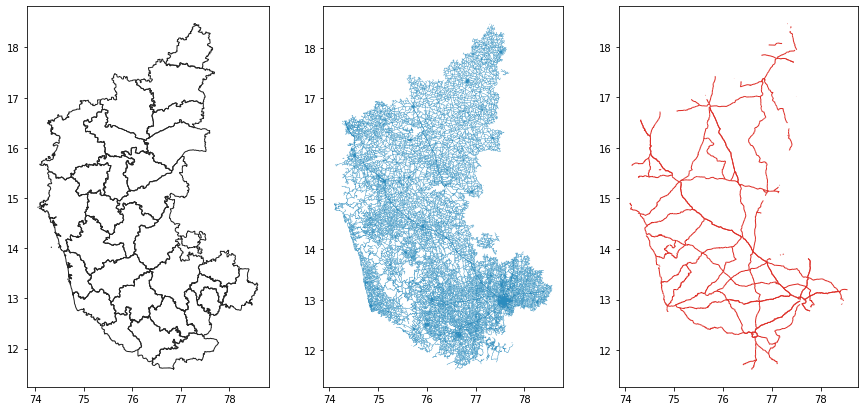<Figure size 432x288 with 0 Axes>

ax0.axis('off')
ax0.set_title('Karnataka Districts', y=-0.1)
ax1.axis('off')
ax2.axis('off')
ax2.set_title('Karnataka National Highways', y=-0.1)
fig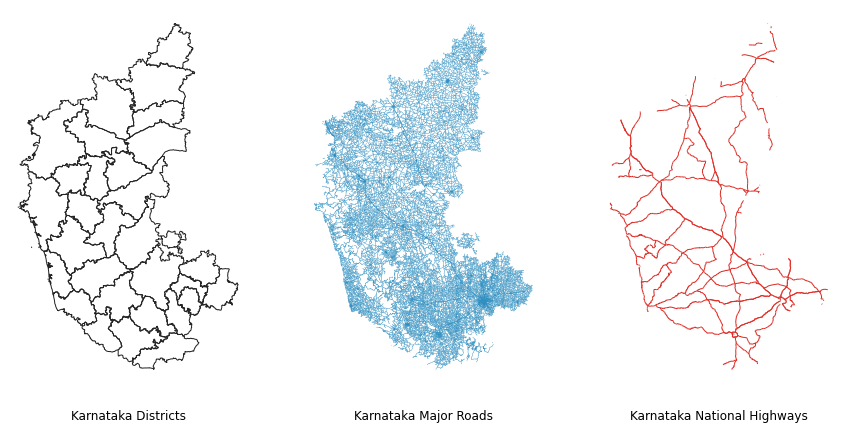output_filename = 'map_layout.png'
output_dir = 'output'
output_path = os.path.join(output_dir, output_filename)

if not os.path.exists(output_dir):
os.mkdir(output_dir)

fig.savefig(output_path, dpi=300)

创建多层地图

fig, ax = plt.subplots()
fig.set_size_inches(10,15)

plt.axis('off')

districts.plot(ax=ax, linewidth=1, facecolor='none', edgecolor='#252525')
national_highways.plot(ax=ax, linewidth=1, color='#de2d26')

output_filename = 'multiple_layers.png'
output_path = os.path.join(output_dir, output_filename)
plt.savefig(output_path, dpi=300)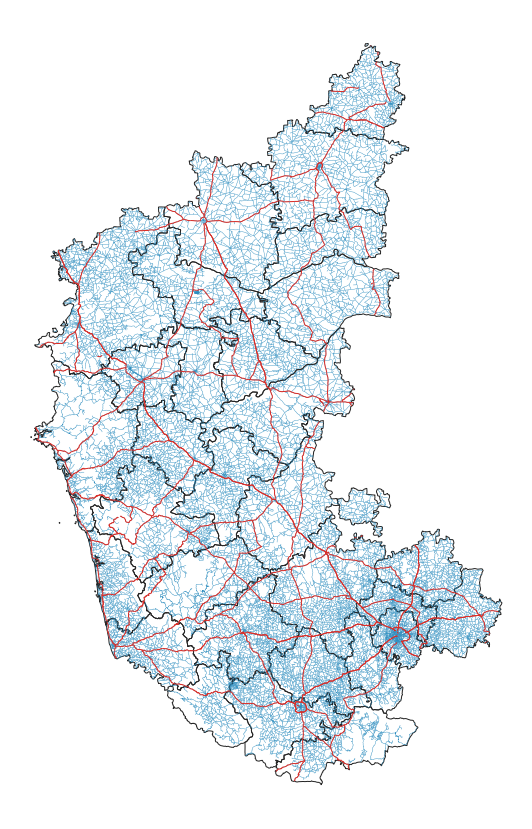标注特征

districts['label_position'] = districts['geometry'].apply(lambda x: x.representative_point().coords[:])
districts['label_position'] = [coords for coords in districts['label_position']]

fig, ax = plt.subplots(figsize=(10, 15))
plt.axis('off')

districts.plot(ax=ax, linewidth=1, facecolor='none', edgecolor='#252525')

for idx, row in districts.iterrows():
plt.annotate(text=row['DISTRICT'], xy=row['label_position'], horizontalalignment='center')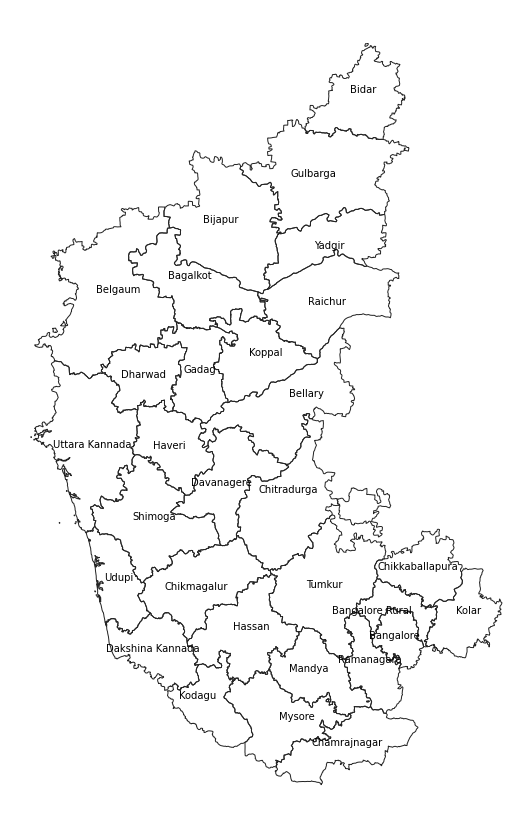使用Xarray

Xarray 是改进版的rasterio，收到pandas等用于处理栅格数据的库的启发。特别适合处理多维度的时序的栅格数据（ multi-dimensional time-series raster datasets）。还和dask紧密结合，支持使用并行计算来将栅格数据处理规模化。

rioxarray是对xarray的扩展，使之更适合用于地理空间栅格数据。可以使用conda-forge或者pip来安装rioxarray.

XArray 和 rioxarray 基础

import os
data_pkg_path = 'data'
srtm_dir = 'srtm'
filename = 'N28E087.hgt'
path = os.path.join(data_pkg_path, srtm_dir, filename)

import rioxarray as rxr

open_rasterio()方法可以用于读取任何rasterio库支持的数据格式。

rds = rxr.open_rasterio(path)

type(rds)
xarray.core.dataarray.DataArray

rds.values
array([[[5217, 5211, 5208, ..., 5097, 5098, 5089],
[5206, 5201, 5200, ..., 5080, 5075, 5069],
[5199, 5194, 5191, ..., 5063, 5055, 5048],
...,
[5347, 5345, 5343, ..., 5747, 5750, 5757],
[5338, 5338, 5336, ..., 5737, 5740, 5747],
[5332, 5331, 5332, ..., 5734, 5736, 5744]]], dtype=int16)

rds.coords
Coordinates:
* band         (band) int64 1
* x            (x) float64 87.0 87.0 87.0 87.0 87.0 ... 88.0 88.0 88.0 88.0
* y            (y) float64 29.0 29.0 29.0 29.0 29.0 ... 28.0 28.0 28.0 28.0
spatial_ref  int64 0

xarray的一个关键特征是可以使用索引查找 index lookup方法来读取数据集的切片。例如，可以使用sel()方法从主数据集中切片，然后得到通道1（Band1）的数据。

band1 = rds.sel(band=1)

print('CRS:', rds.rio.crs)
print('Resolution:', rds.rio.resolution())
print('Bounds:', rds.rio.bounds())
print('Width:', rds.rio.width)
print('Height:', rds.rio.height)
CRS: EPSG:4326
Resolution: (0.0002777777777777778, -0.0002777777777777778)
Bounds: (86.99986111111112, 27.999861111111112, 88.00013888888888, 29.000138888888888)
Width: 3601
Height: 3601

合并栅格数据

import rioxarray as rxr
from rioxarray.merge import merge_arrays

srtm_path = os.path.join(data_pkg_path, 'srtm')
all_files = os.listdir(srtm_path)
output_filename = 'merged.tif'
output_dir = 'output'
output_path = os.path.join(output_dir, output_filename)

datasets = []
for file in all_files:
path = os.path.join(srtm_path, file)
datasets.append(rxr.open_rasterio(path))

merged = merge_arrays(datasets)

merged.rio.to_raster(output_path)

使用Matplotlib进行栅格数据可视化

xarray的投图函数其实也是基于流行的matplotlib库。

%matplotlib inline
import matplotlib.pyplot as plt

fig, axes = plt.subplots(1, 4)
fig.set_size_inches(15,3)
plt.tight_layout()
for index, dataset in enumerate(datasets):
ax = axes[index]
dataset.plot(ax=ax, cmap='Greys_r')
ax.axis('off')
filename = all_files[index]
ax.set_title(filename)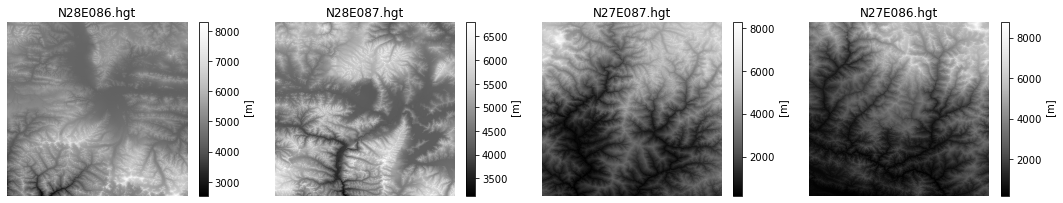fig, ax = plt.subplots()
fig.set_size_inches(12, 10)
merged.plot(ax=ax, cmap='Greys_r')
ax.set_title('merged')
plt.show()